## Friday, January 04, 2019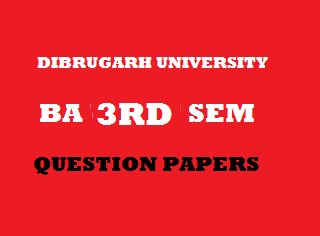2015
(November)
MATHEMATICS
(Major)
Course: 301
[Analysis – I (Real Analysis)]
Full Marks: 80
Pass Marks: 32 (Backlog)/24 (2014 onwards)
Time: 3 hours
The figures in the margin indicate full marks for the questions
GROUP – A
(Differential Calculus)
(Marks: 35)

1. (a) If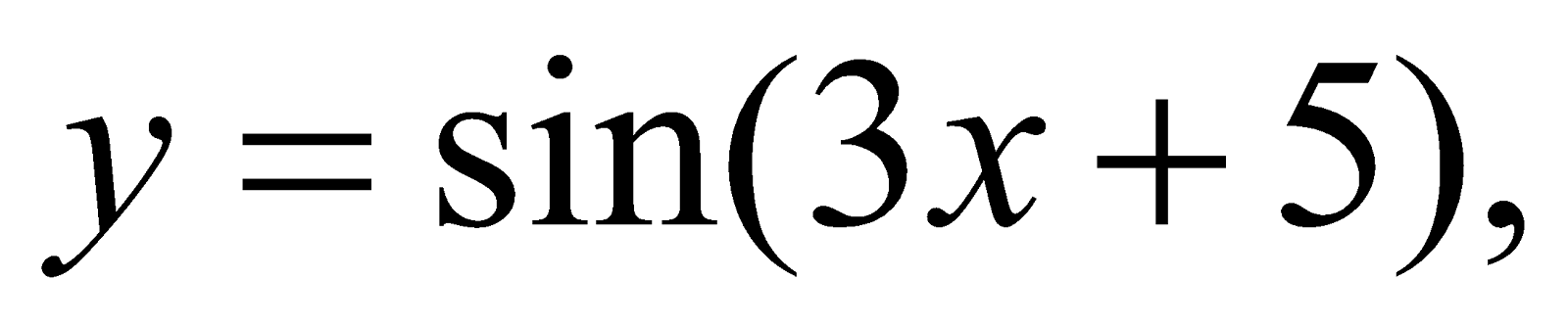then write the value of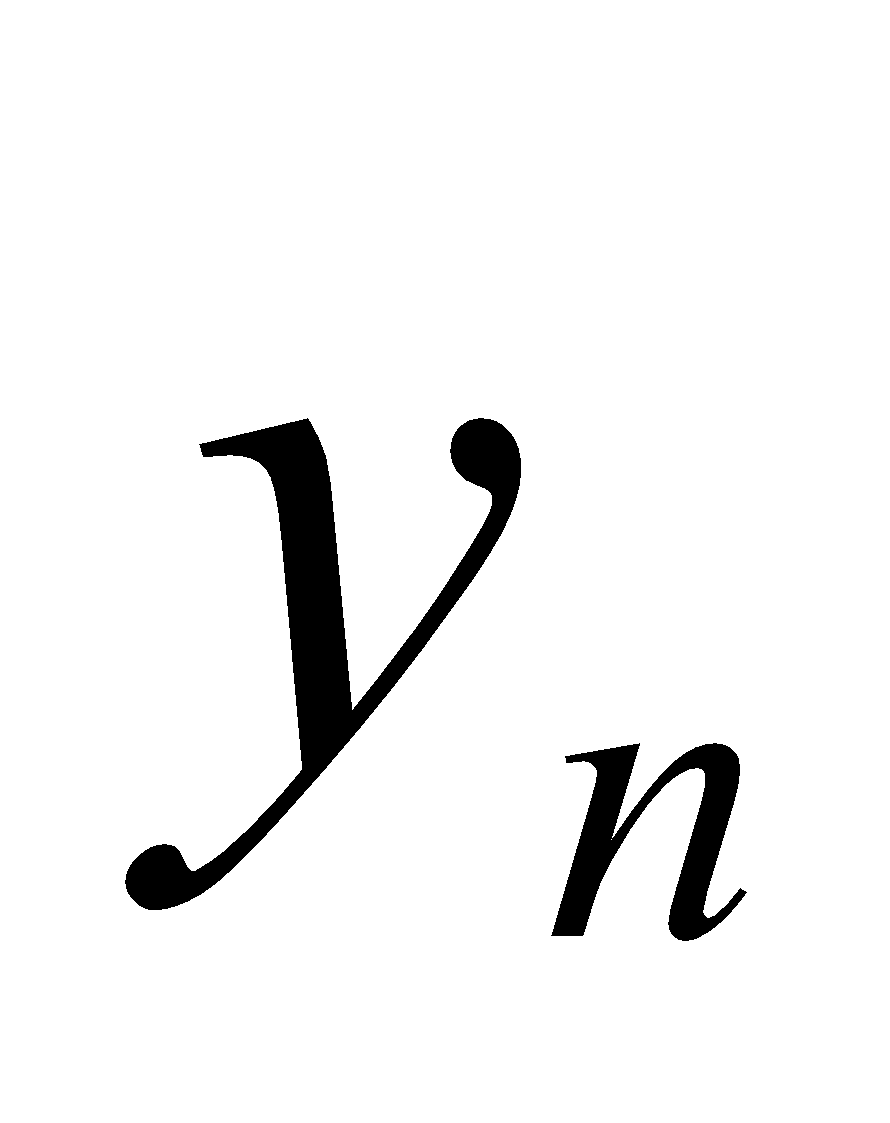. 1
(b) Evaluate: 2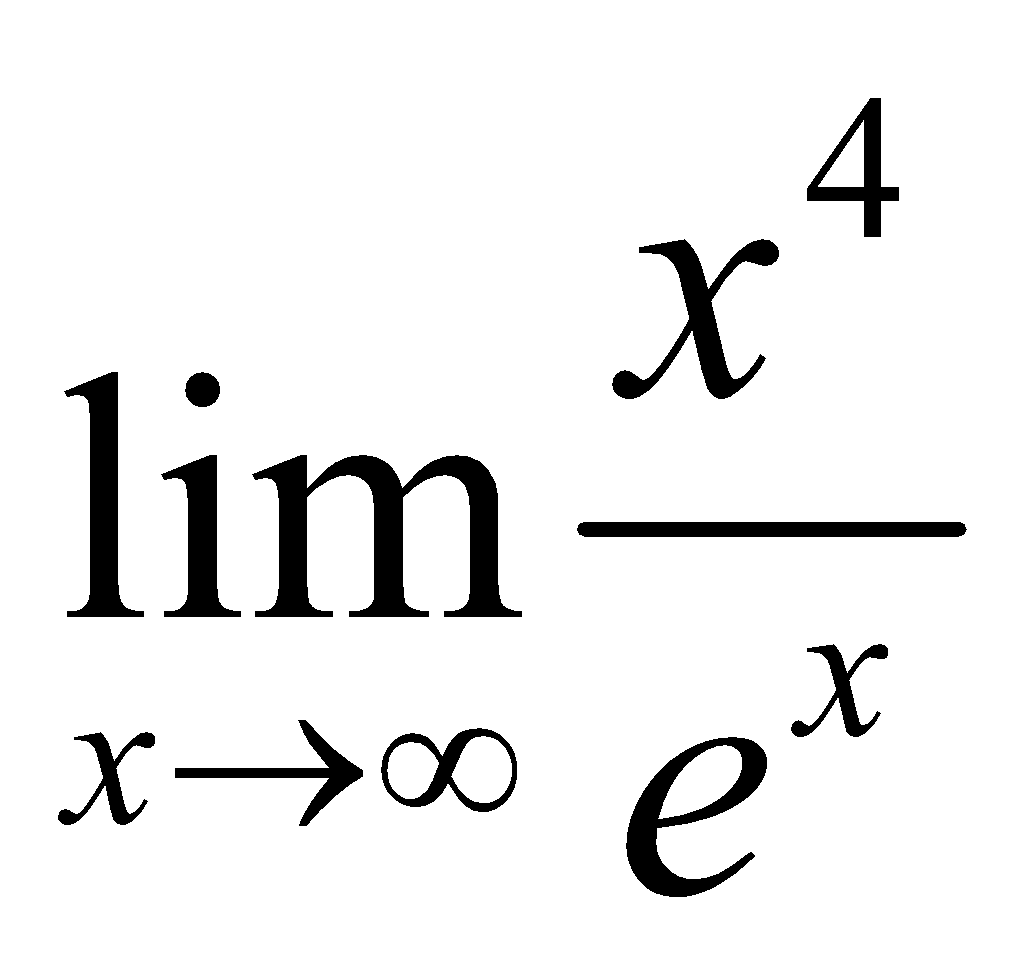(c) Find the length of the subnormal to the curve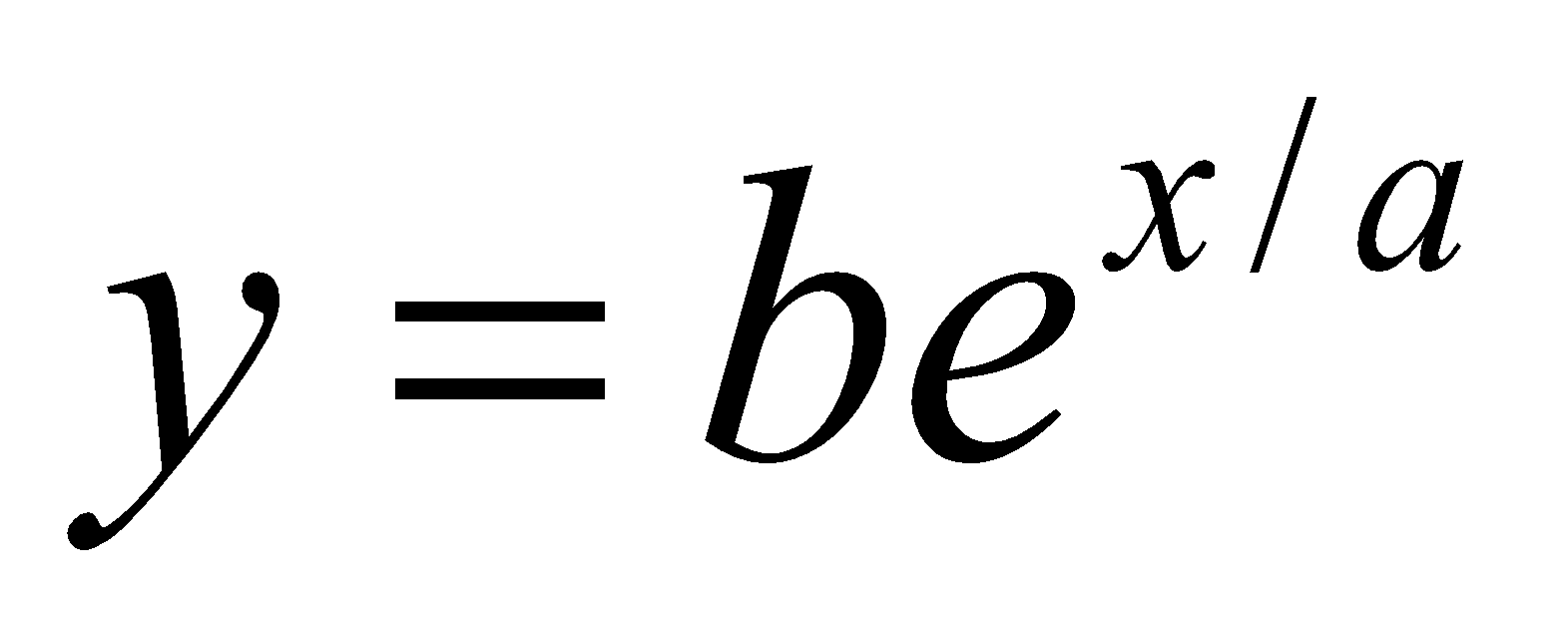at the point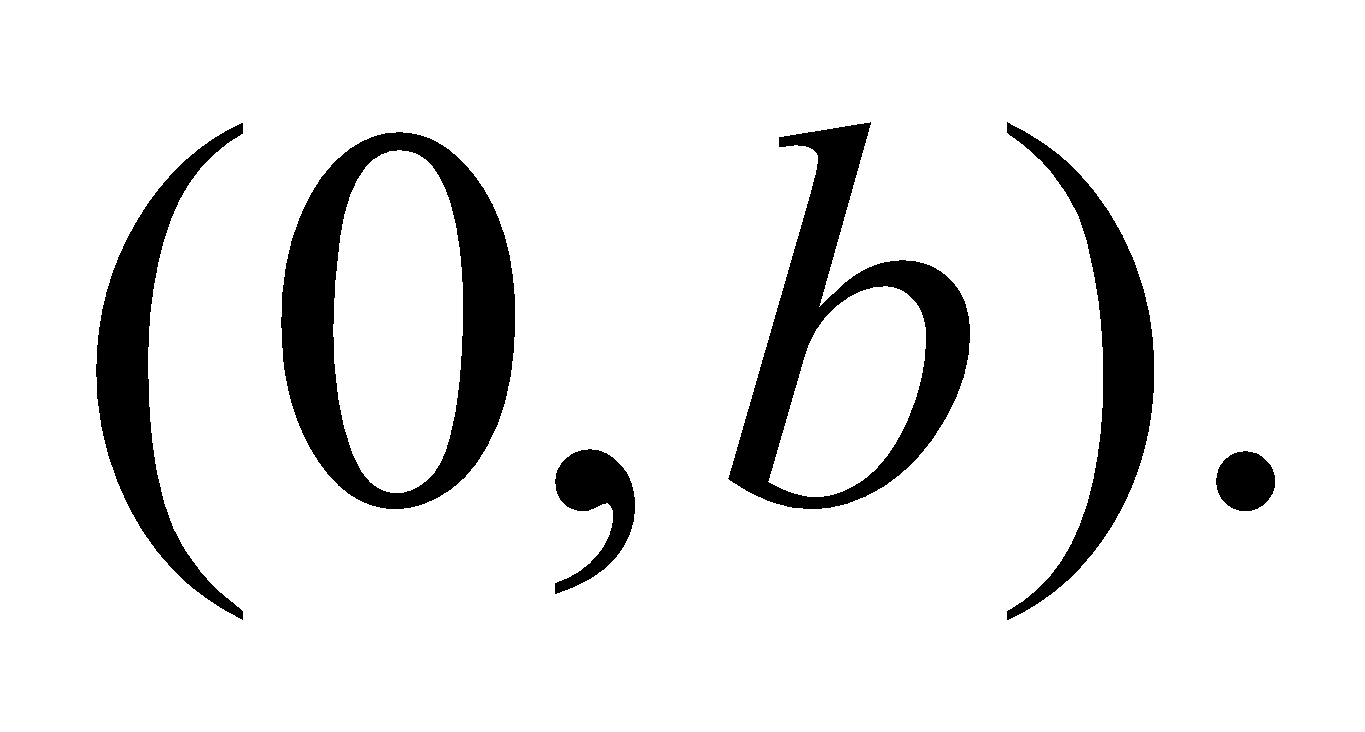3
(d) If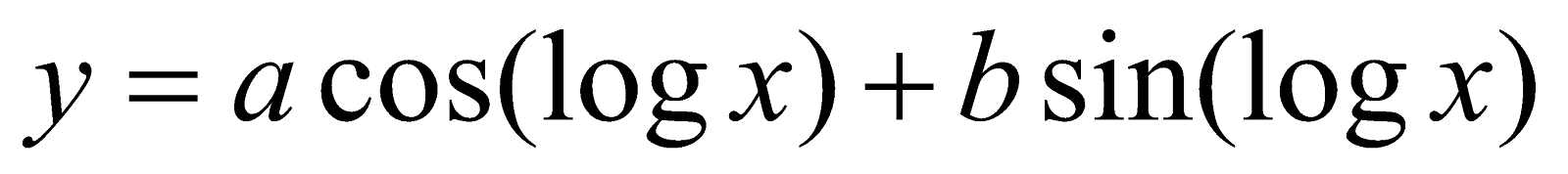, then show that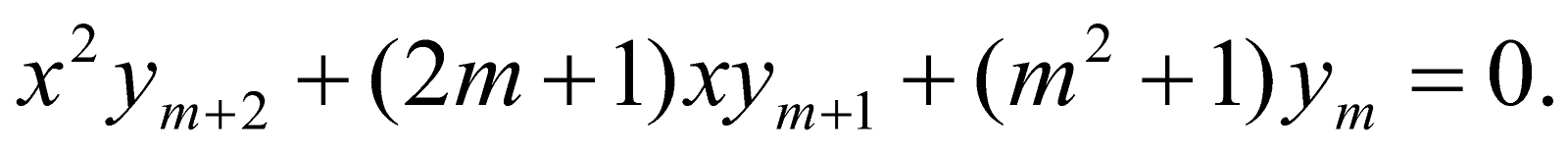4
Or
Find the radius of curvature at the point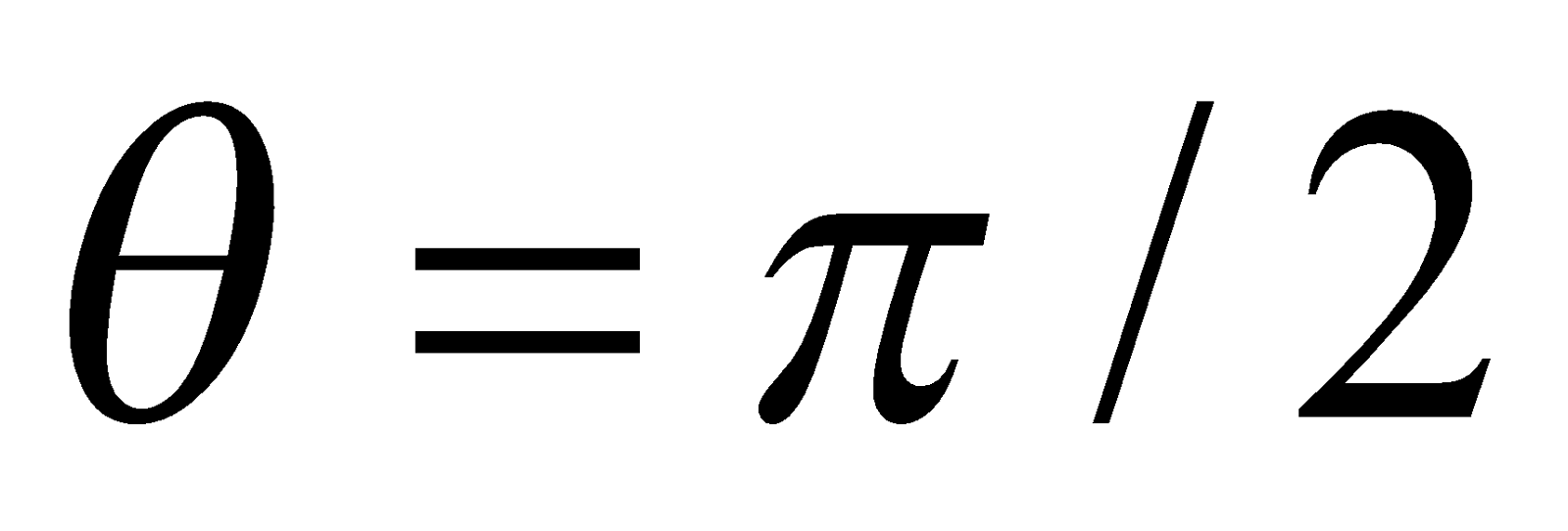on the cycloid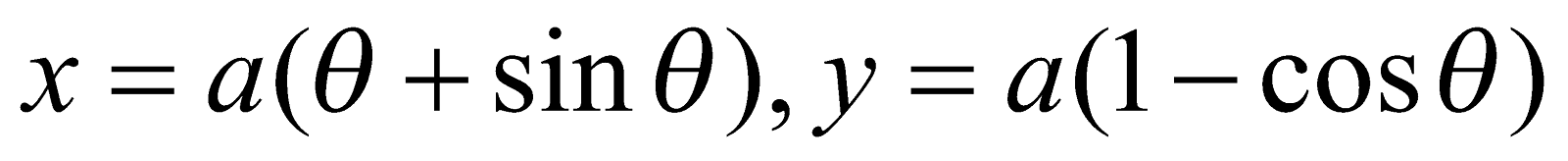2. (a) Write the statement of Darboux’s theorem. 1
(b) Show that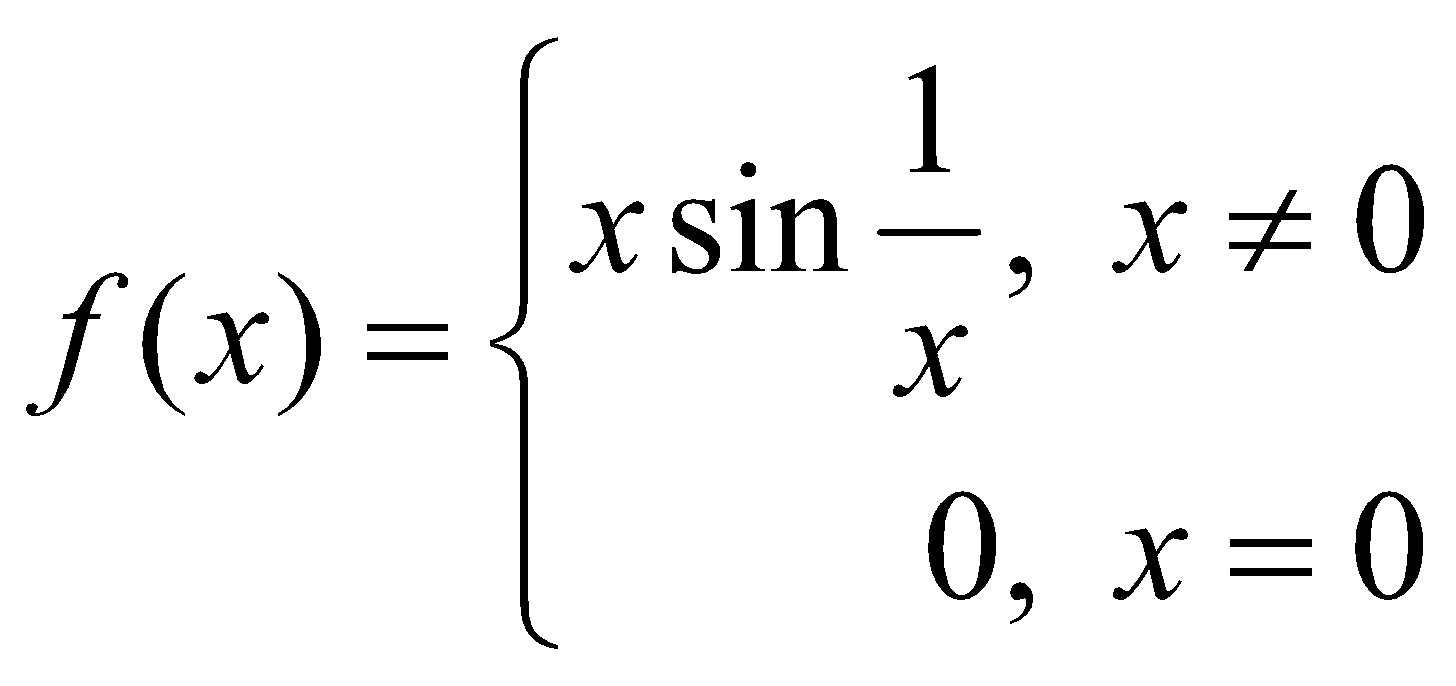is continuous at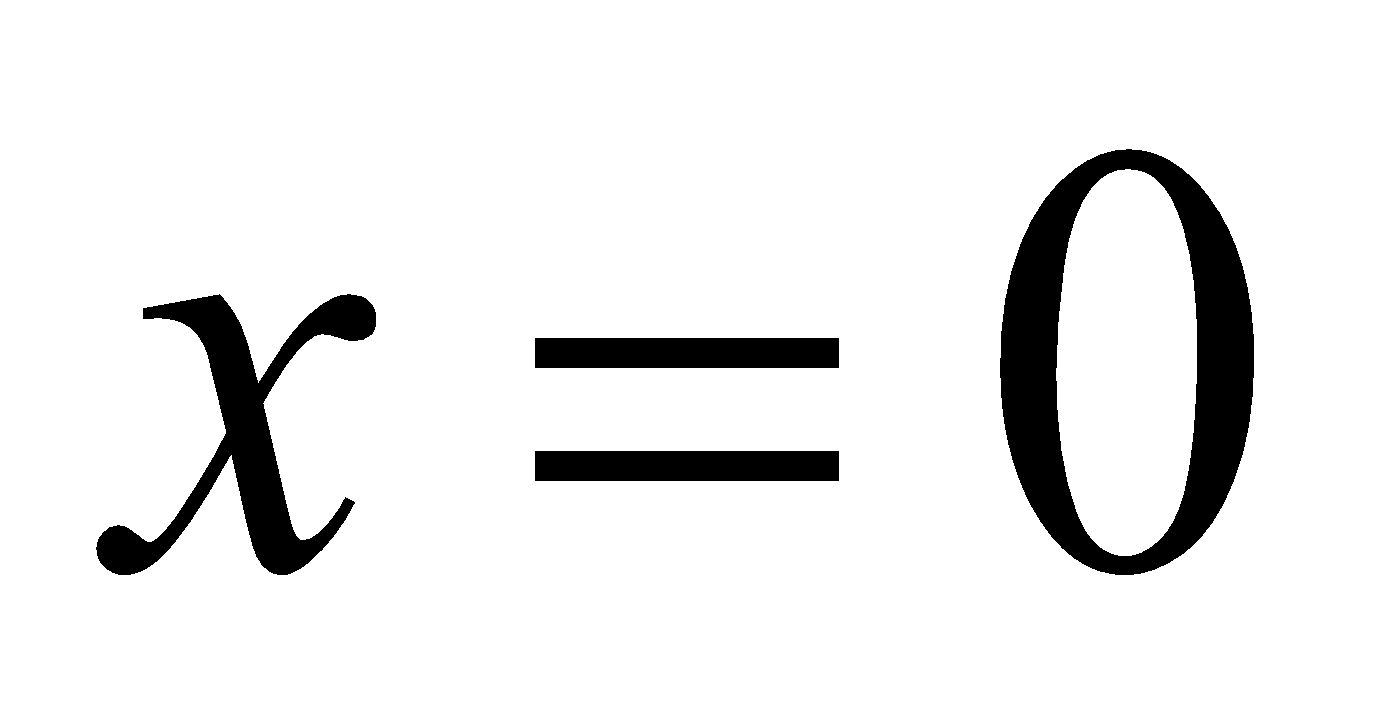2
(c) Show that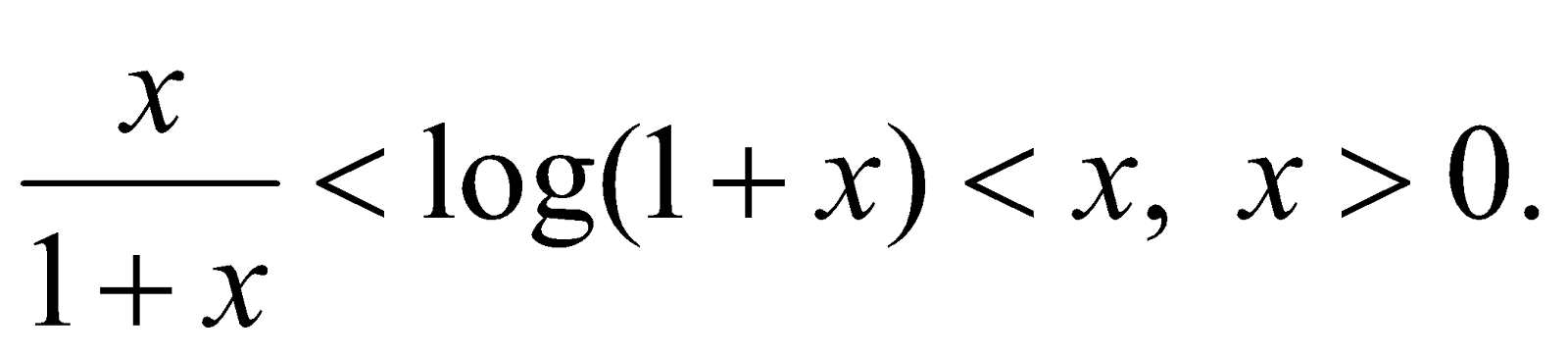4
Or
Expand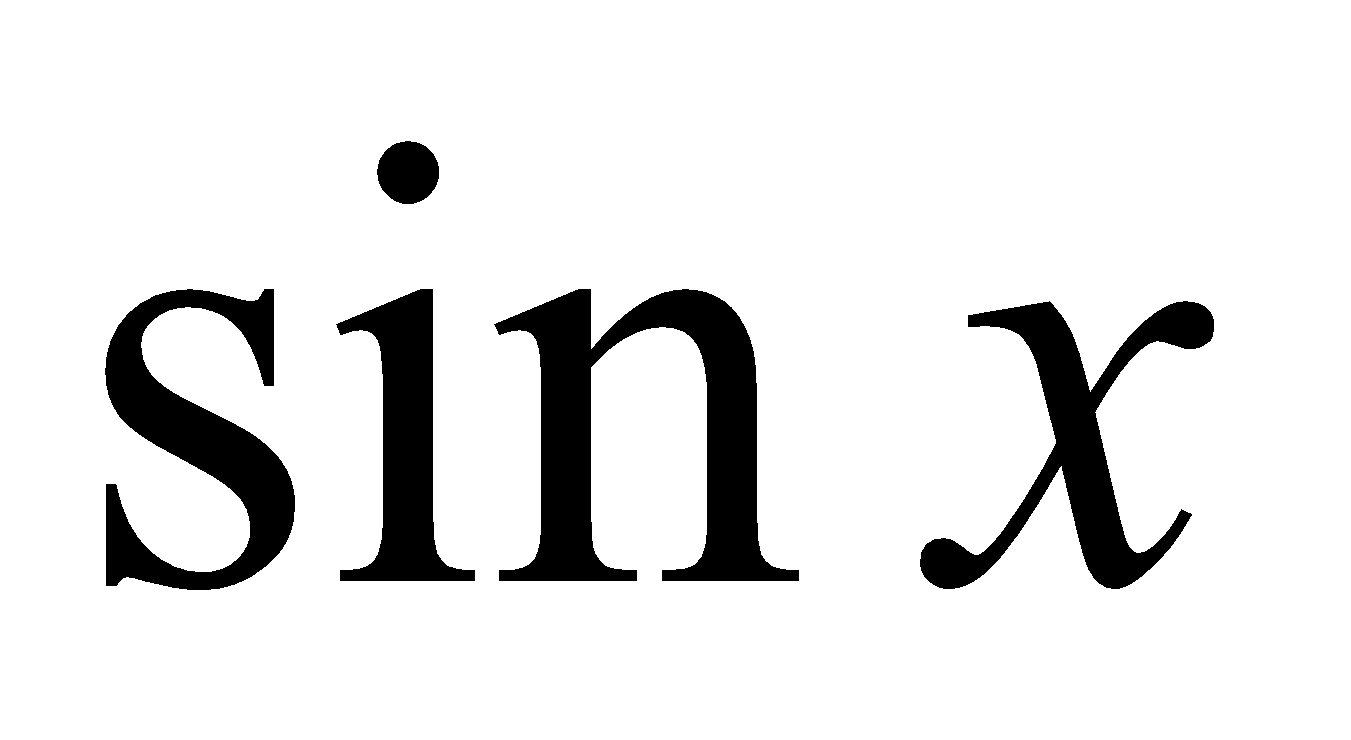in an infinite series using Maclaurin’s series.
(d) Verify Rolle’s Theorem for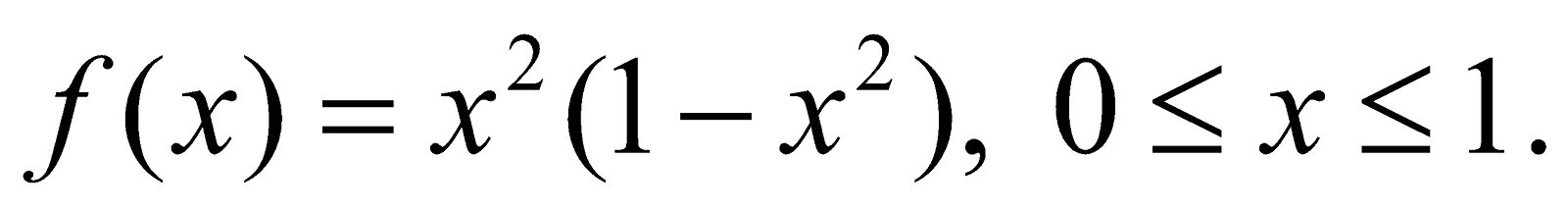3
3. (a) State the Euler’s Theorem on homogeneous function of two variables. 1
(b) If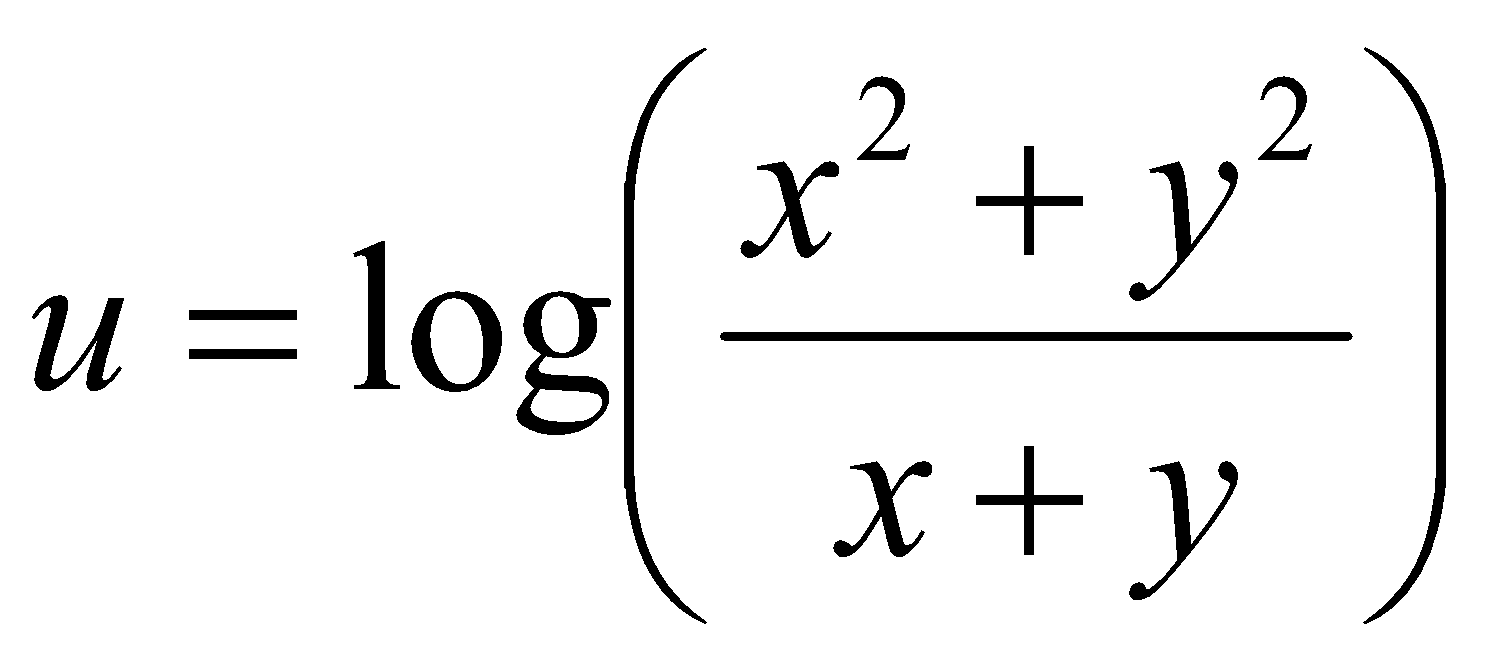then show that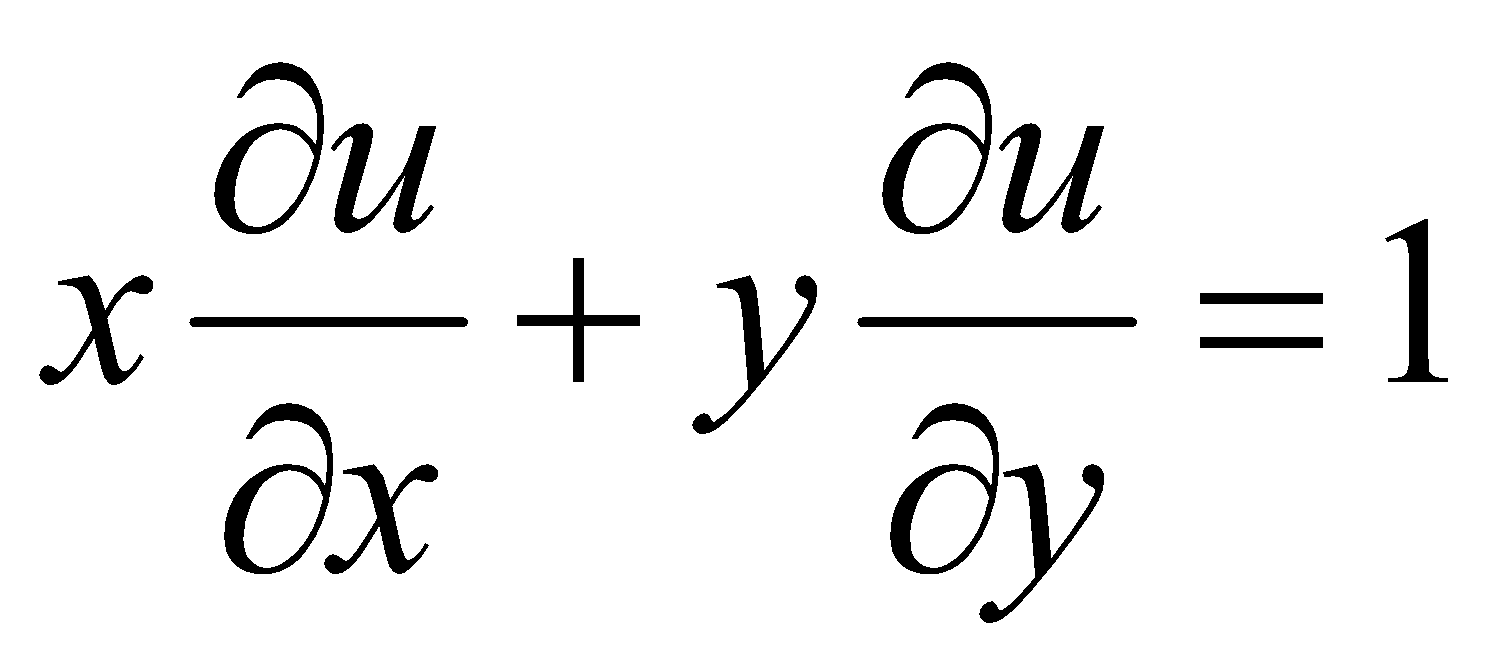4
Or
If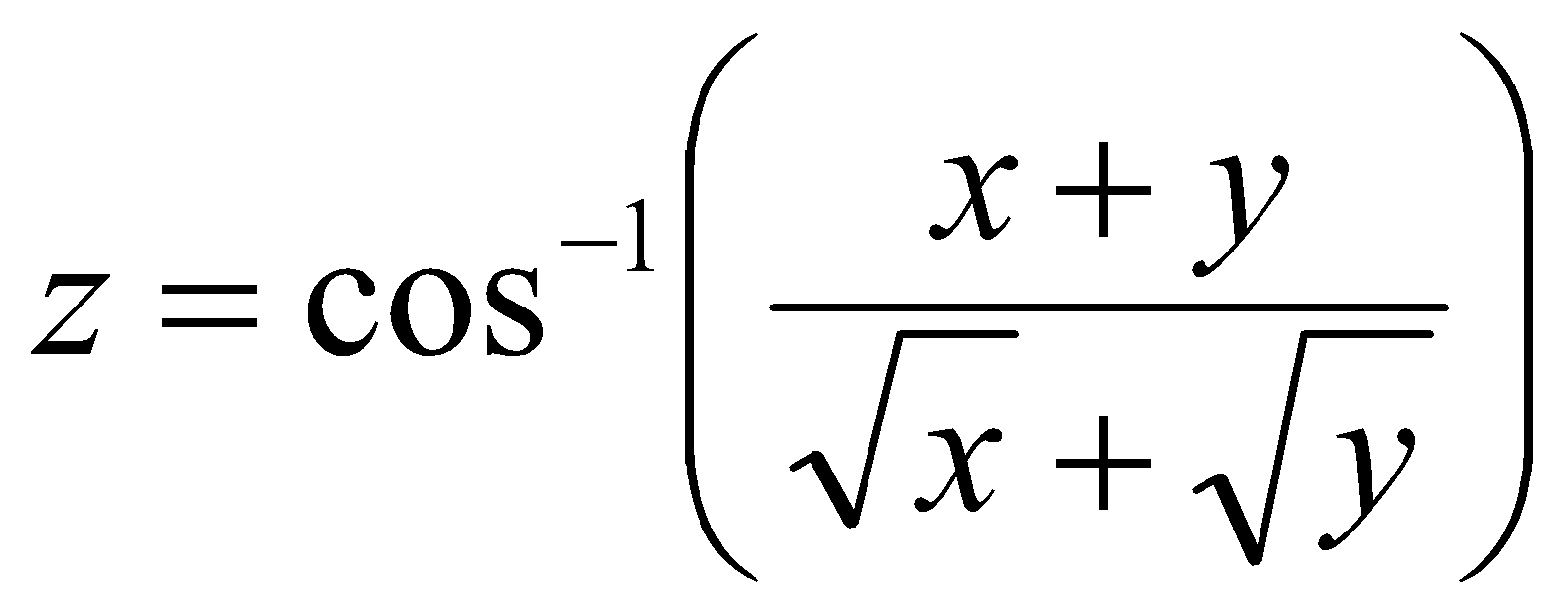then show that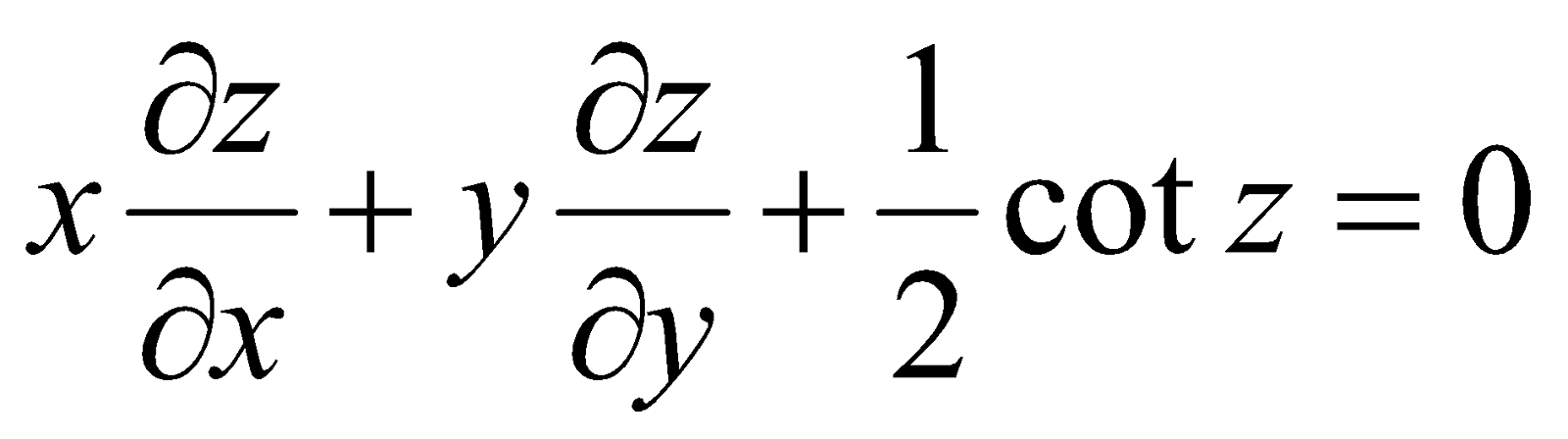4. (a) Write the statement of Schwartz’s theorem. 1
(b) if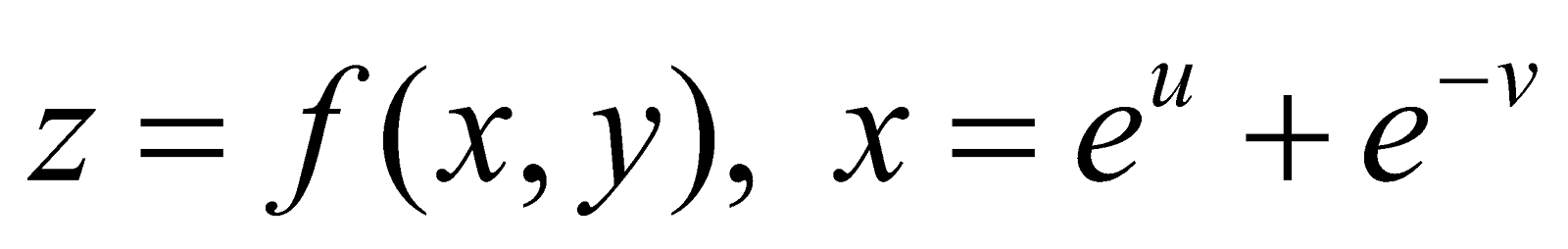and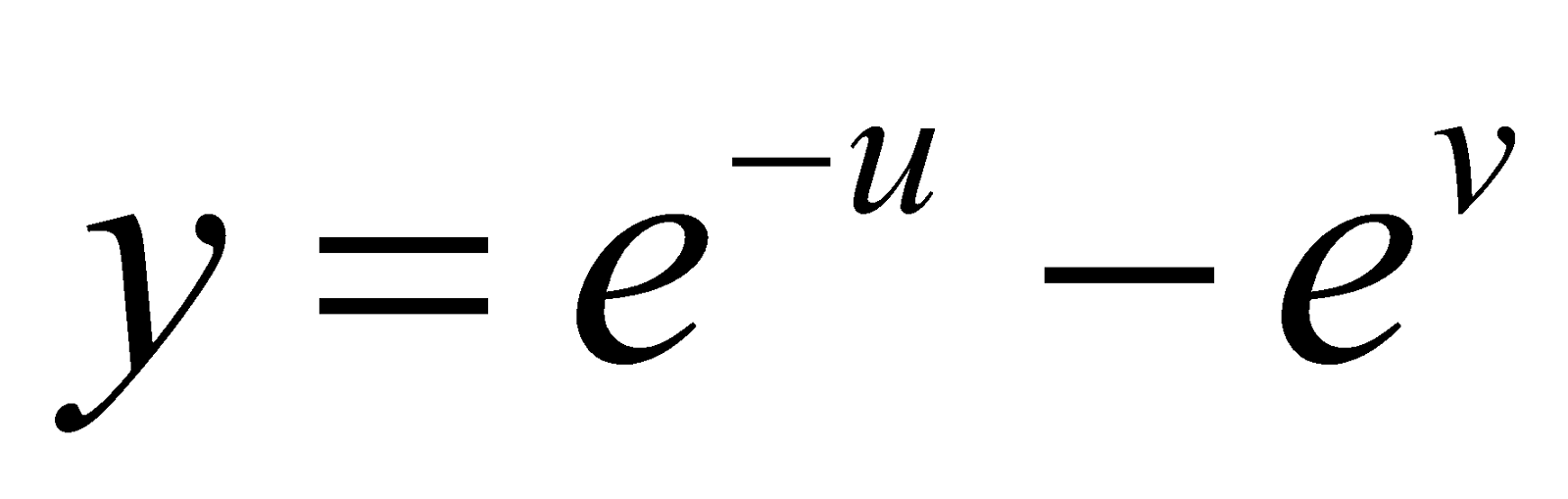, then show that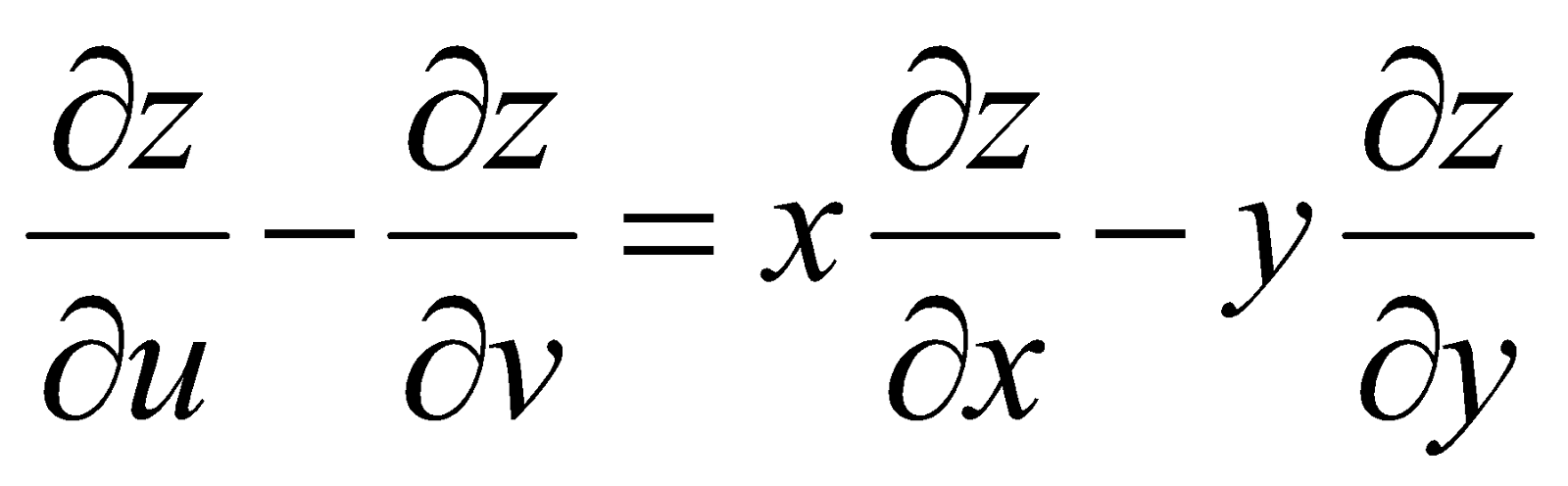4
(c) If z is a function of x and y and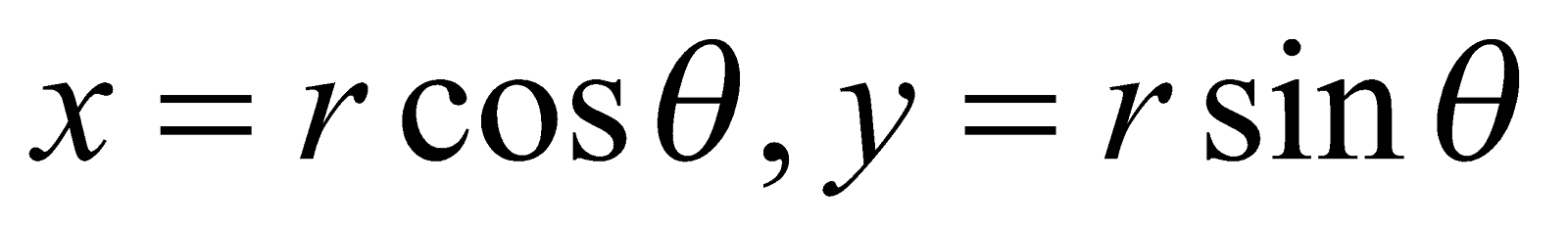, then prove that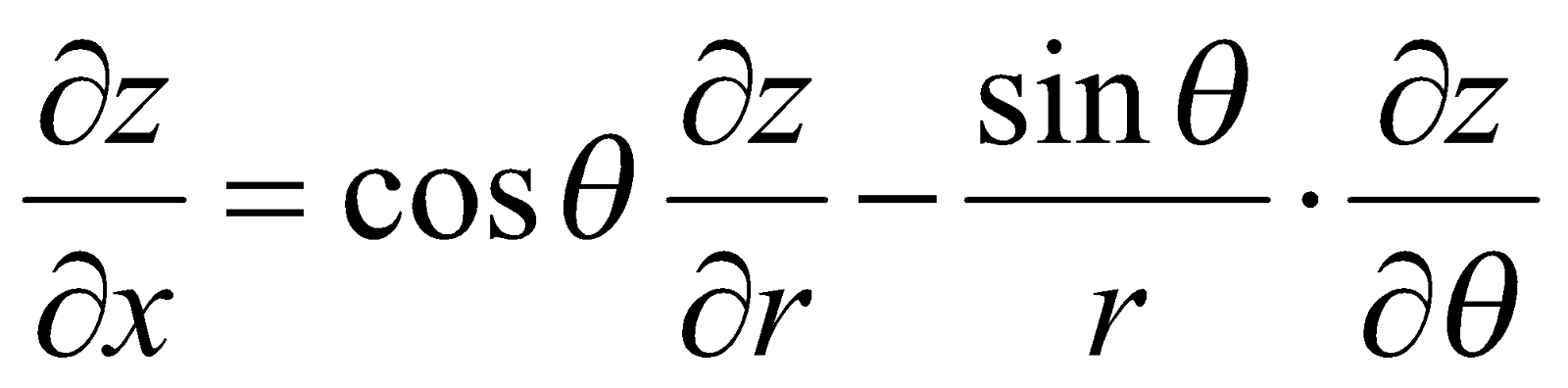5
Or
Prove that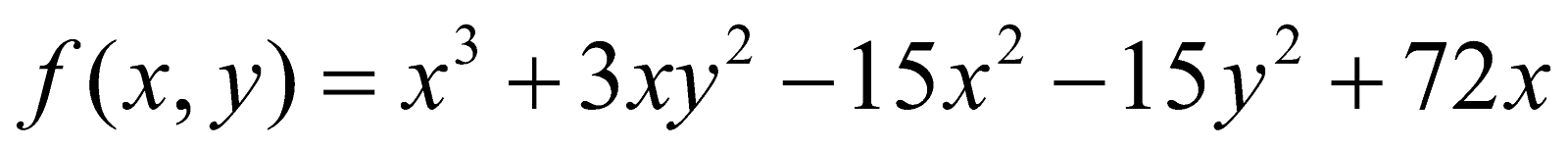has a minimum value at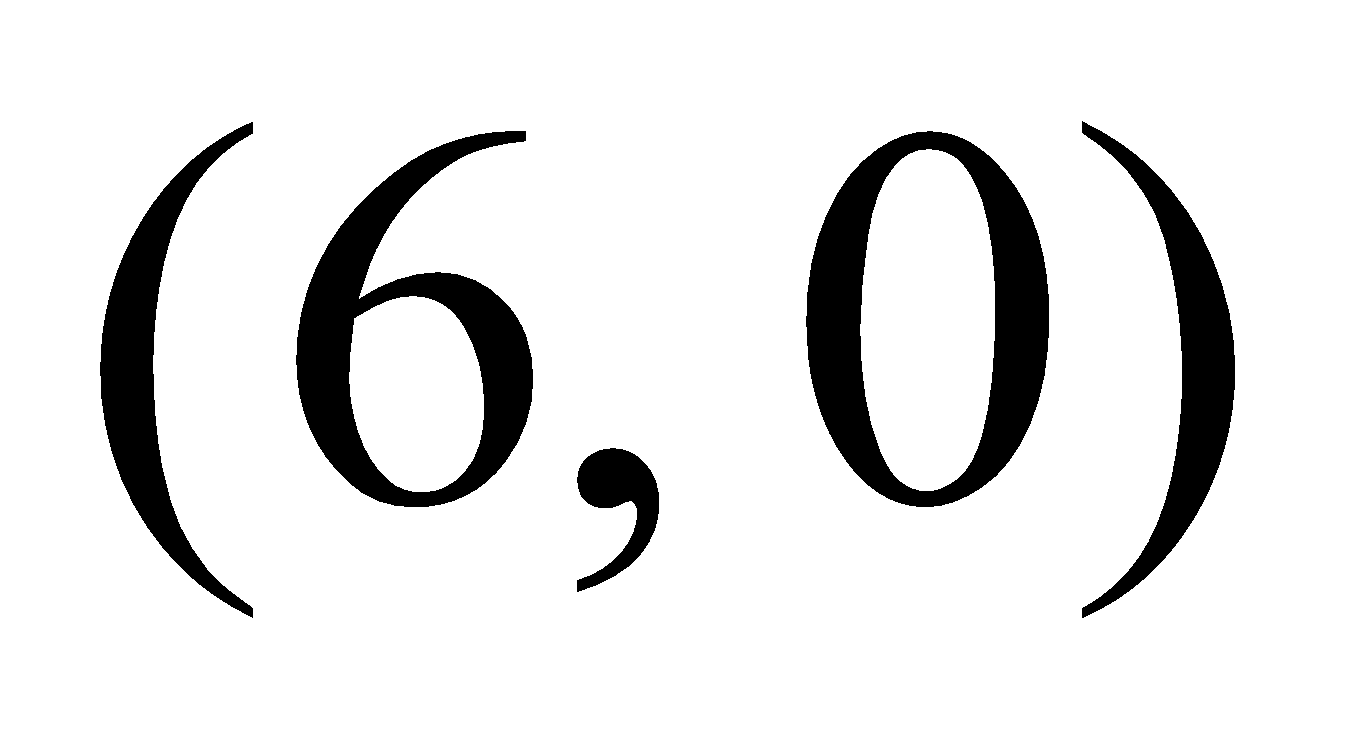.

GROUP – B
(Integral Calculus)
(Marks: 20)

5. (a) Write the value of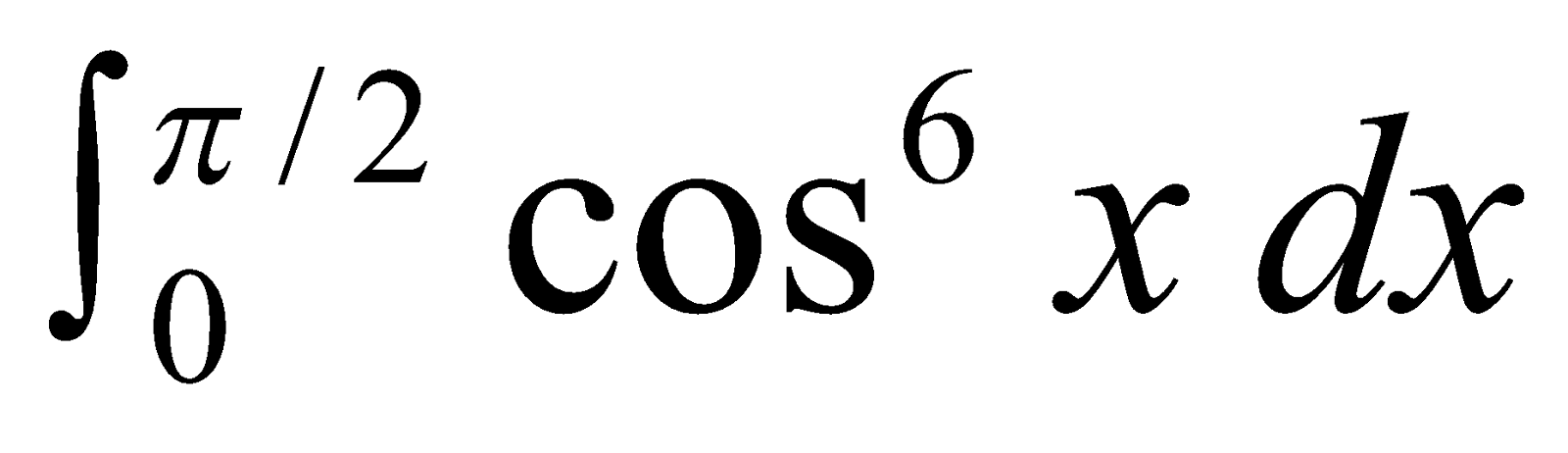1
(b) Prove that 2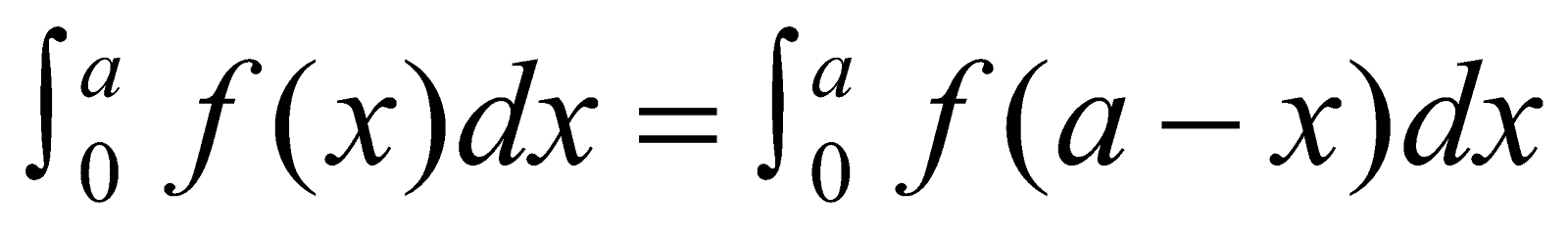(c) Prove that 3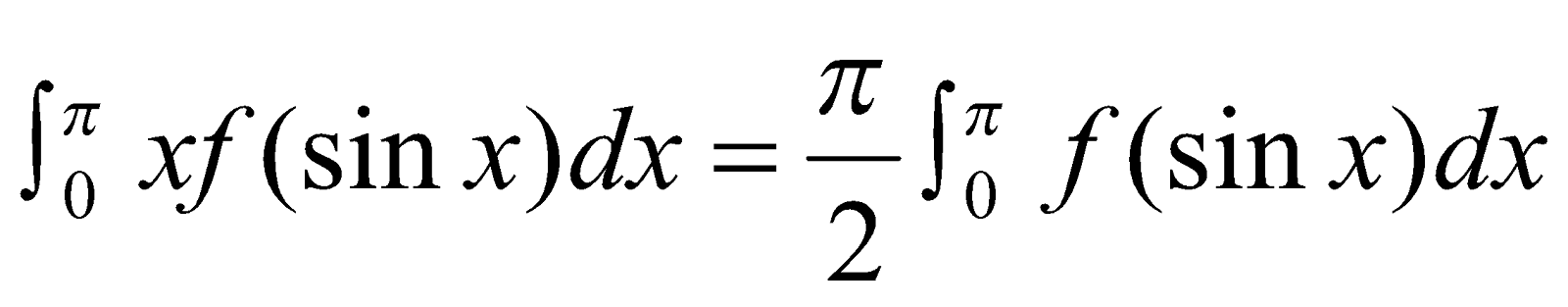(d) Show that 4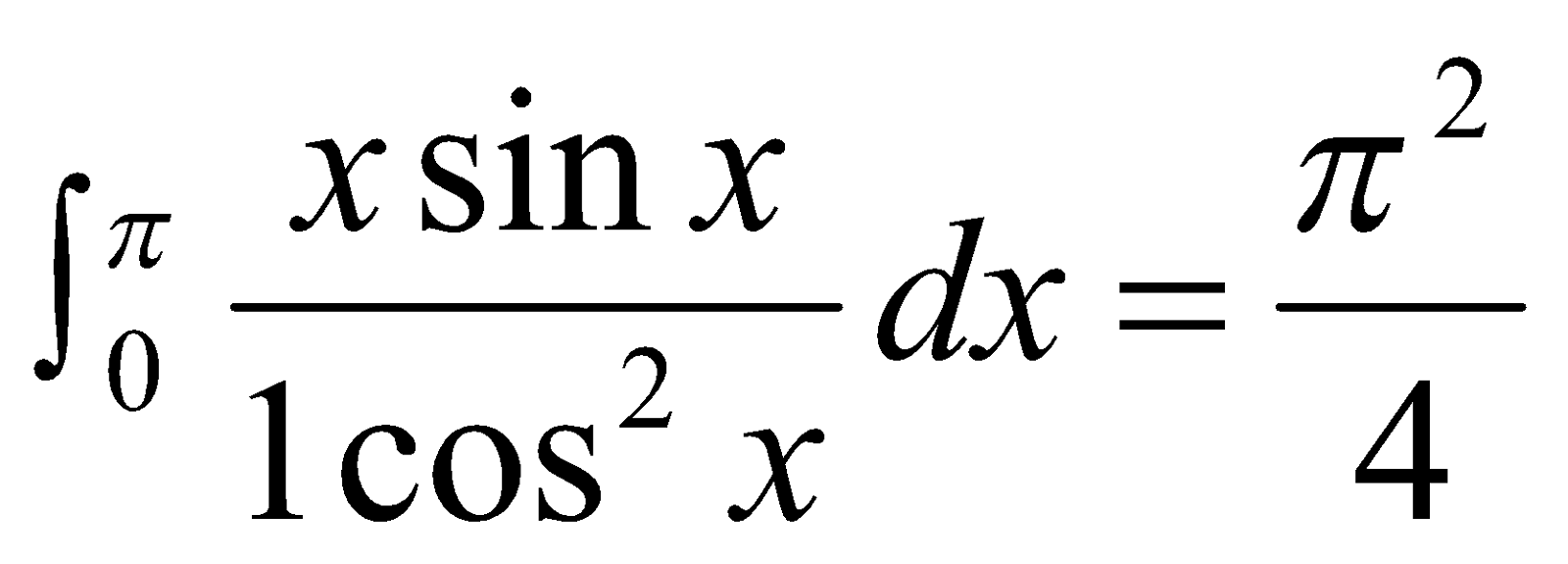Or
Using reduction formula, evaluate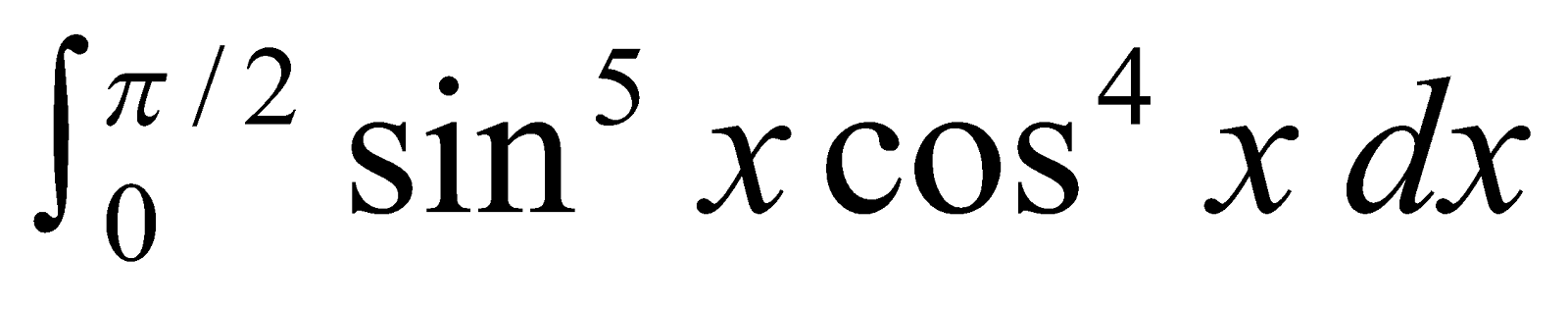6. (a) Write the formula for length of an arc between two points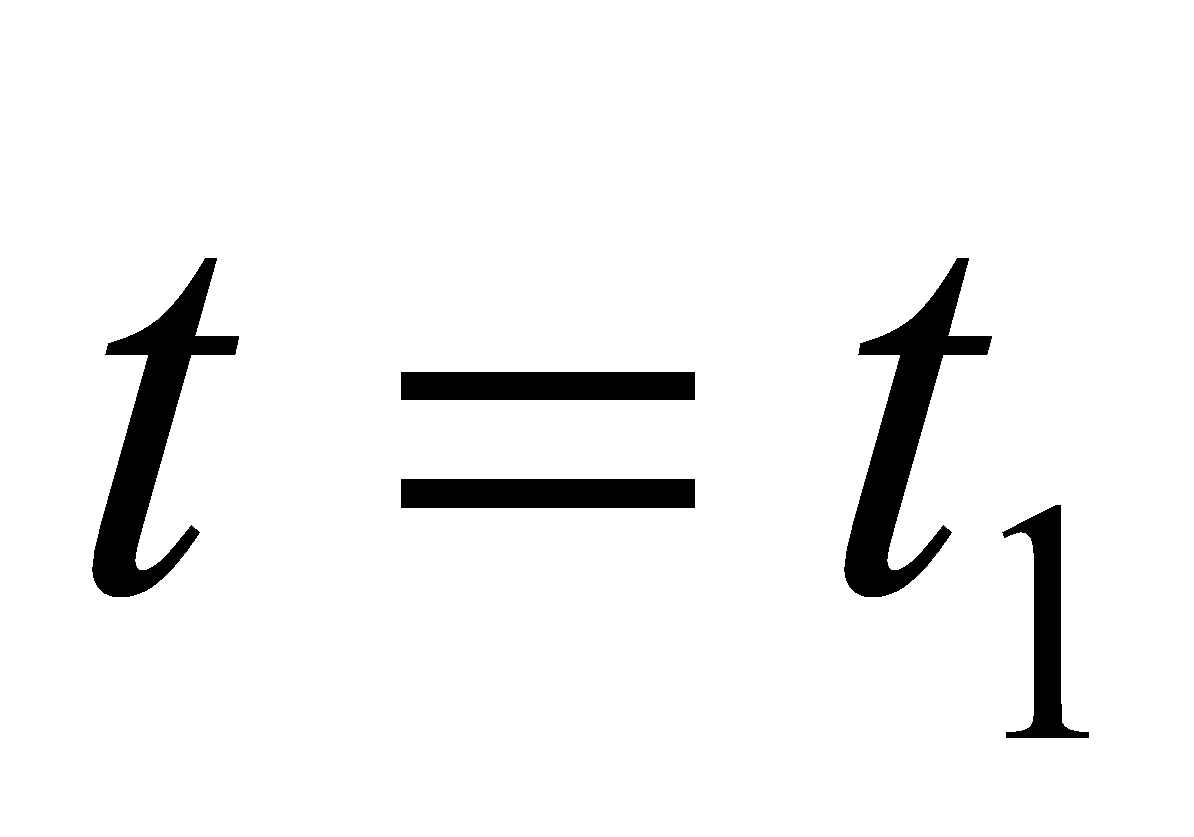and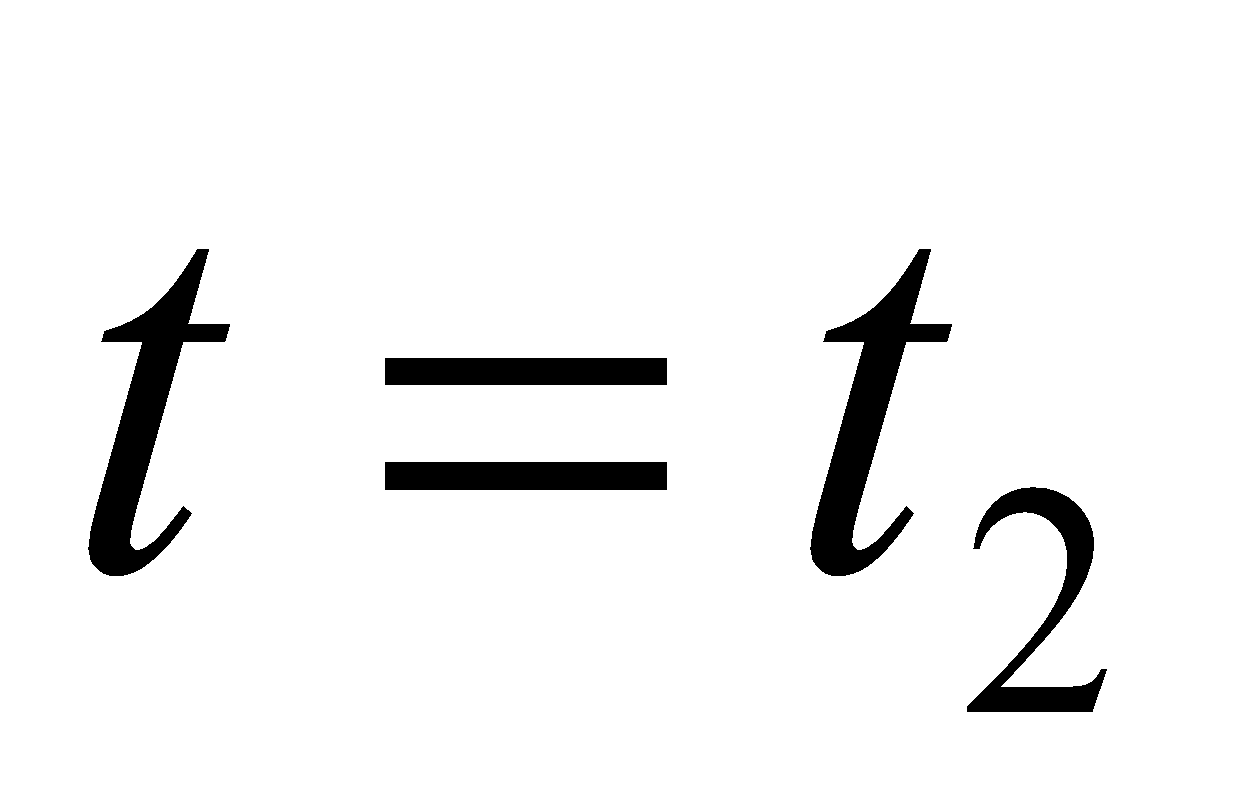when the curve is given in parametric form. 1
(b) Find the length of the arc of the curve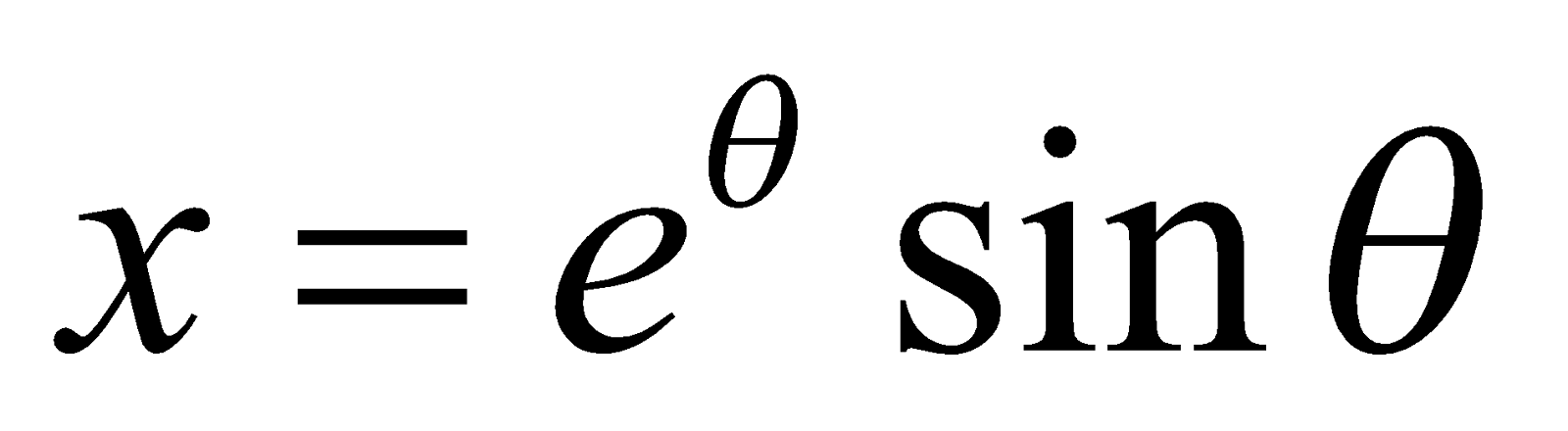and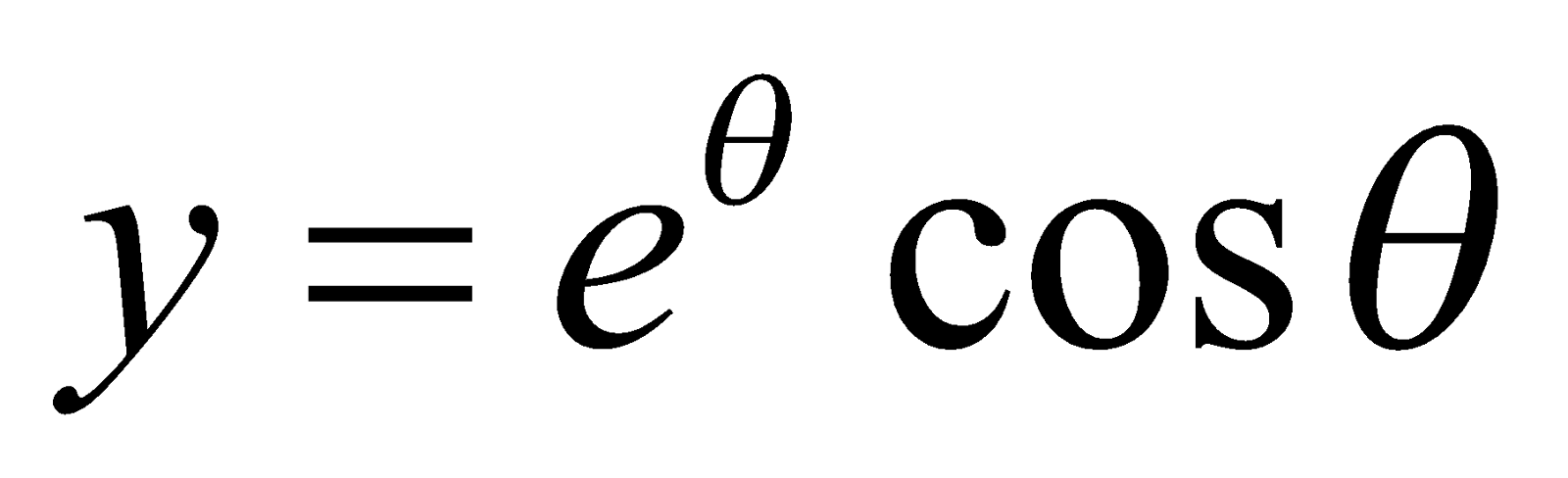from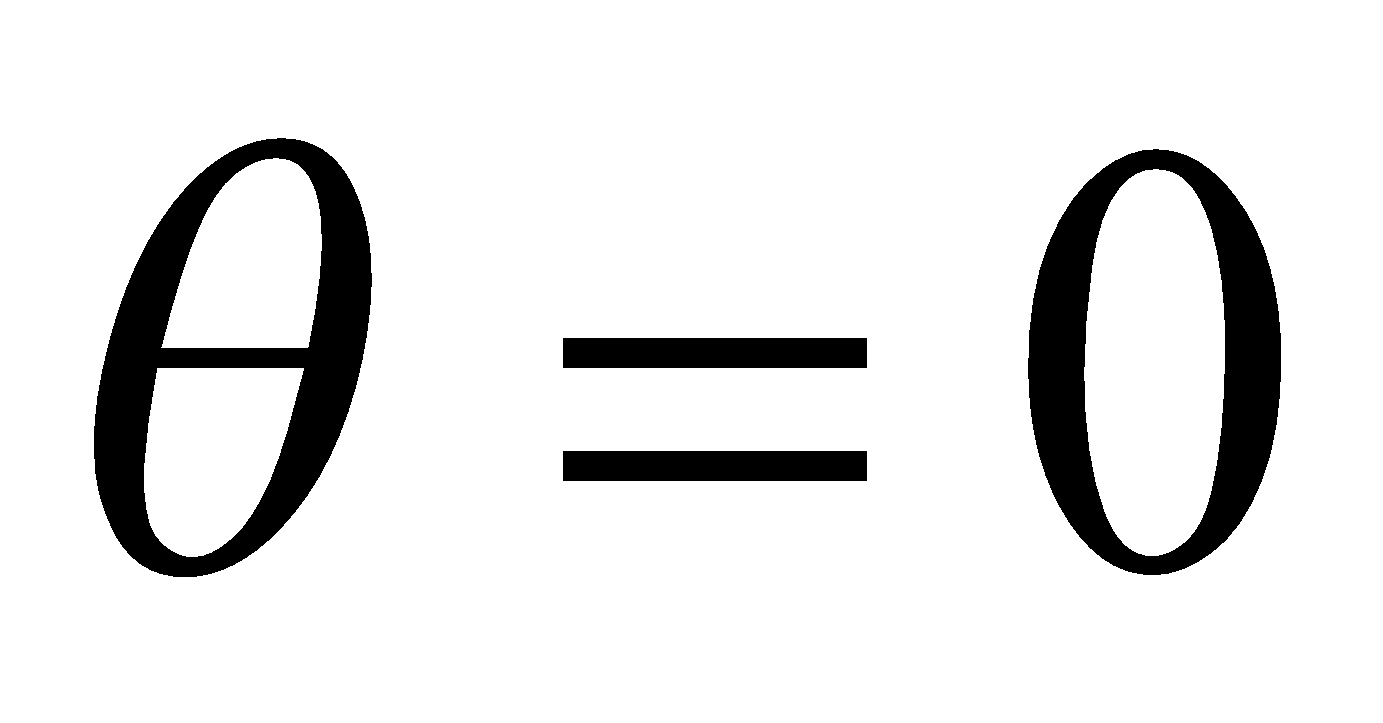to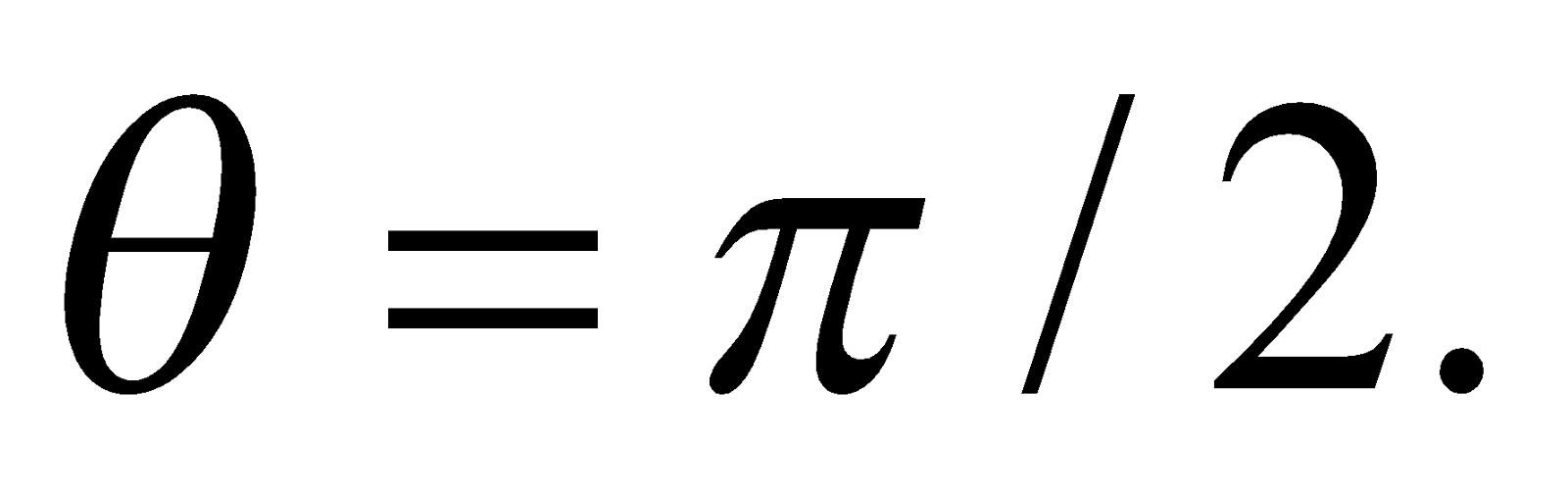4
Or
Find the perimeter of the cardioids(c) Find the volume and surface area of the solid of revolution formed by rotation of the parabola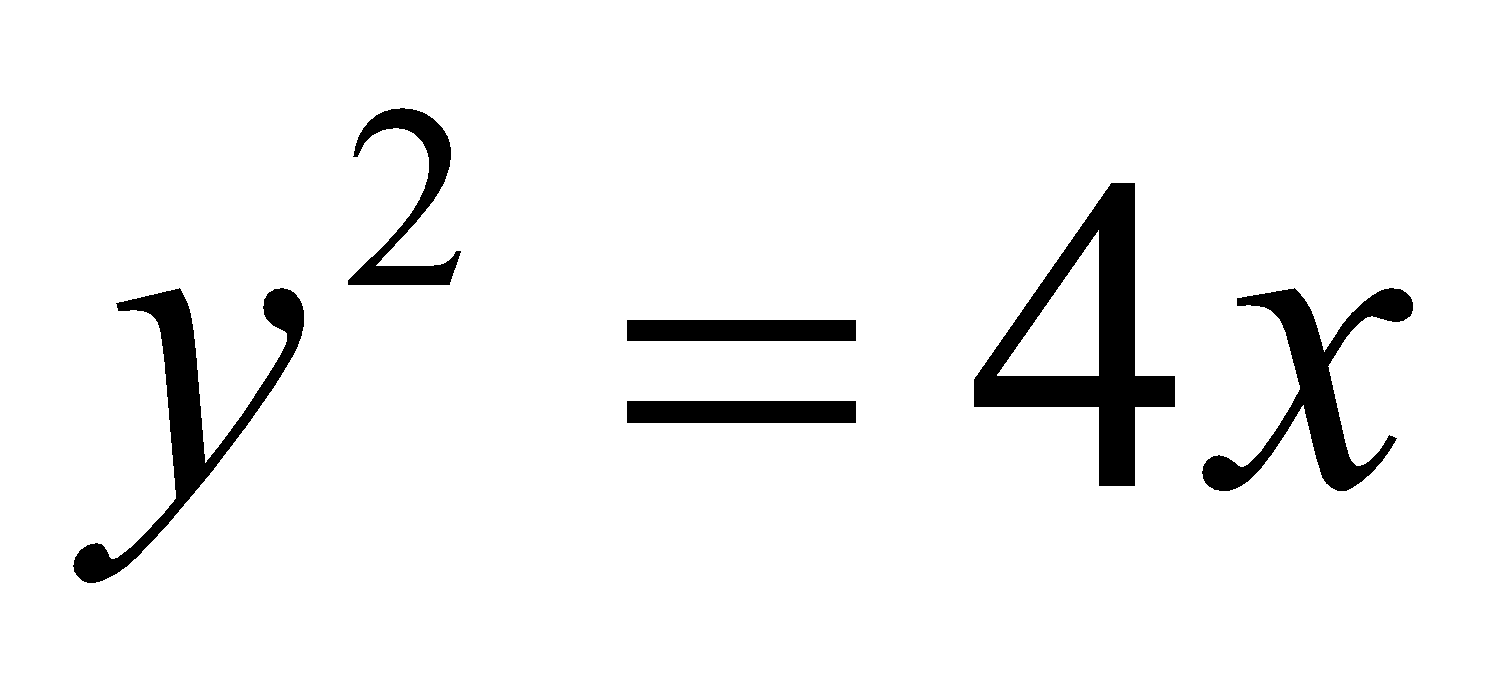about x-axis and bounded by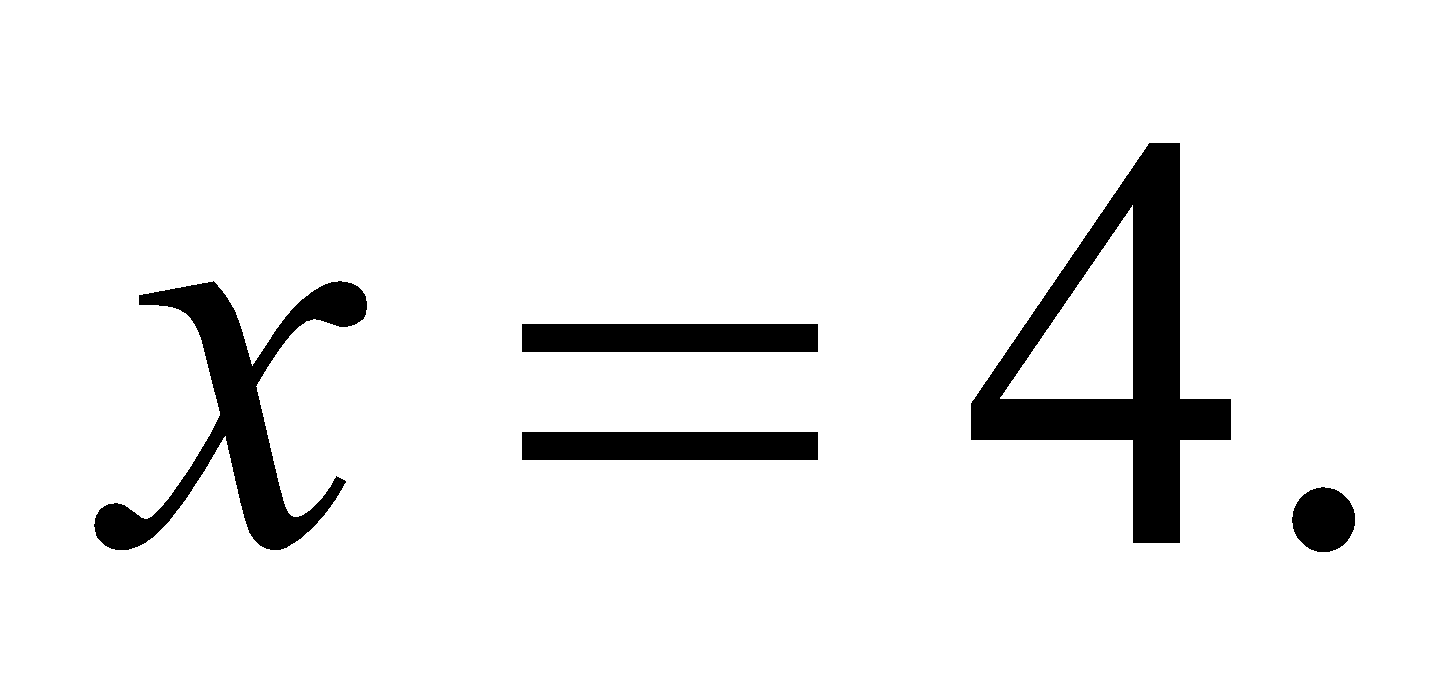5
Or
Find the volume and surface of the solid of revolution of the ellipse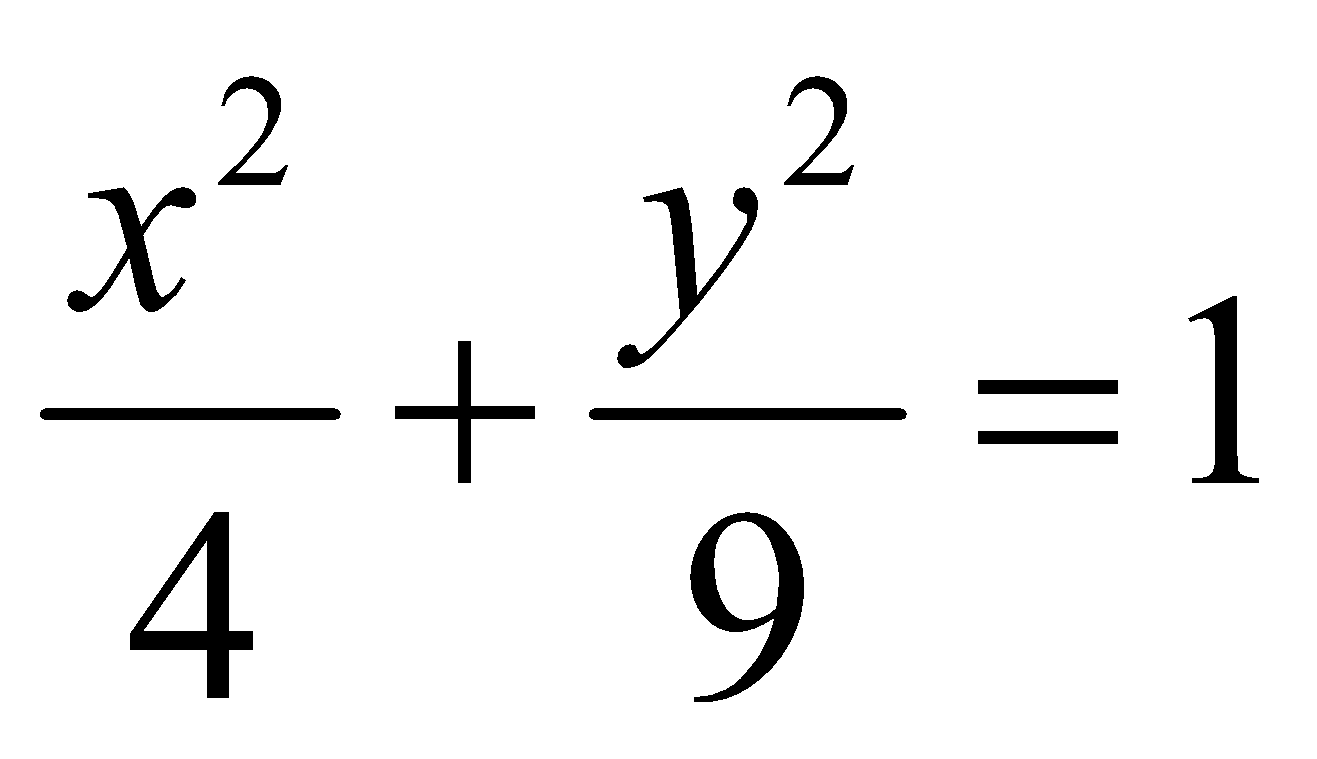GROUP – C
(Riemann Integral)
(Marks: 25)
7. (a) Every bounded function defined on an interval [a, b] is Riemann integrable. State True or False. 1
(b) Prove that a constant function is always Riemann integrable. 3
(c) State and prove the necessary and sufficient condition for a function to be Riemann integrable. 4
Or
Prove that is a function is monotonic on [a, b], then it is Riemann integrable on [a, b].
8. (a) Define primitive of a function. 1
(b) If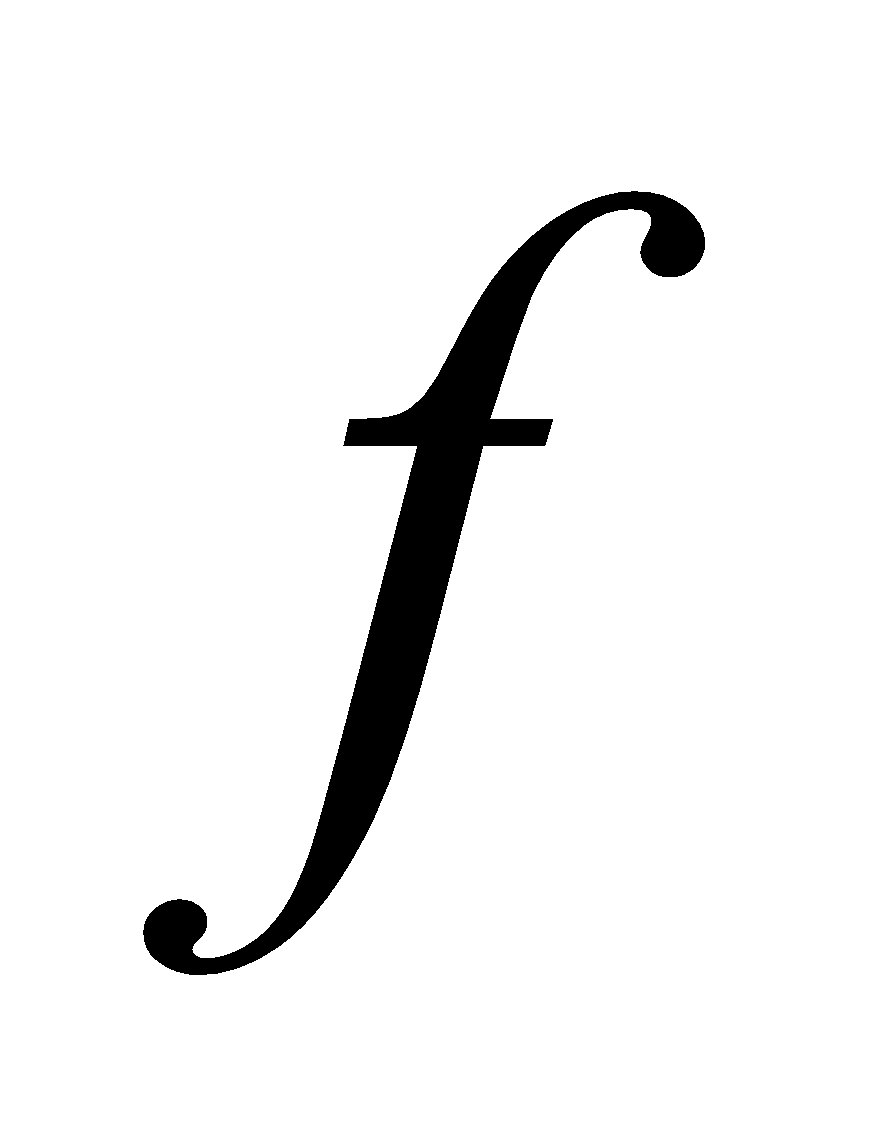is bounded and integrable in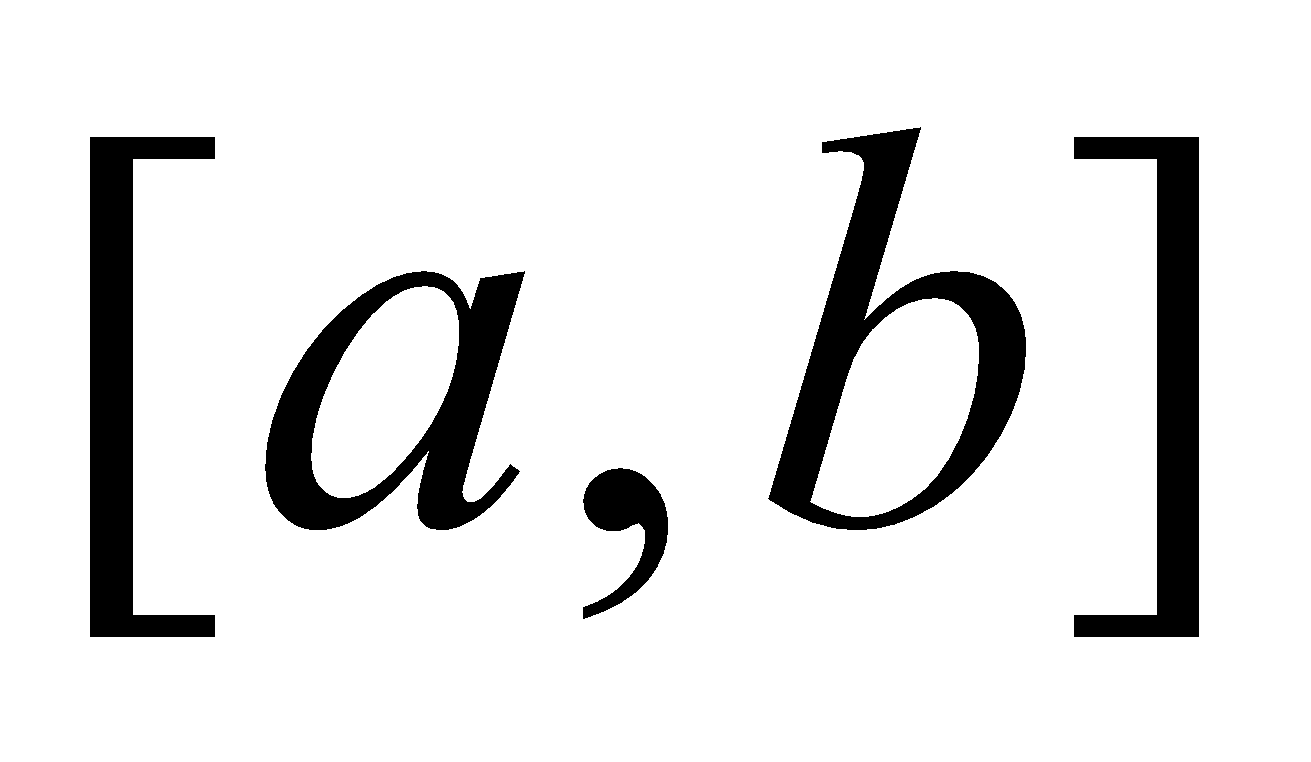, and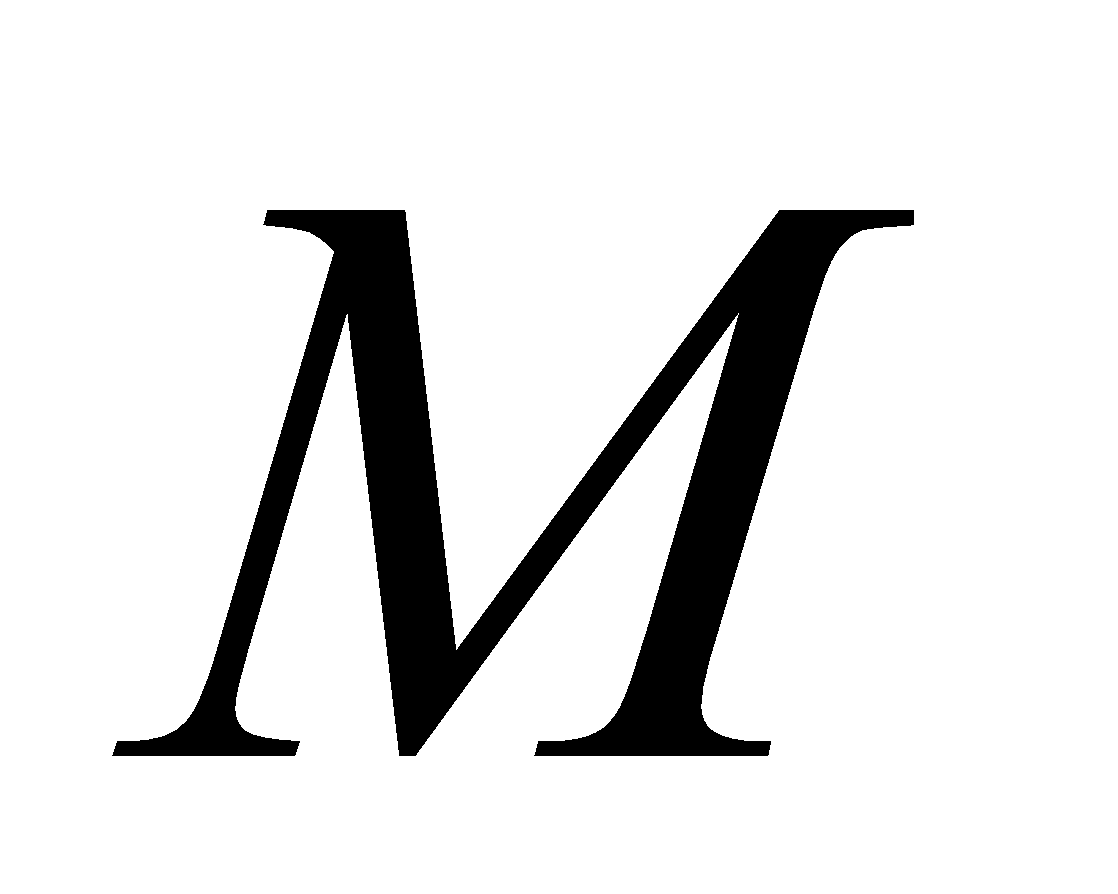and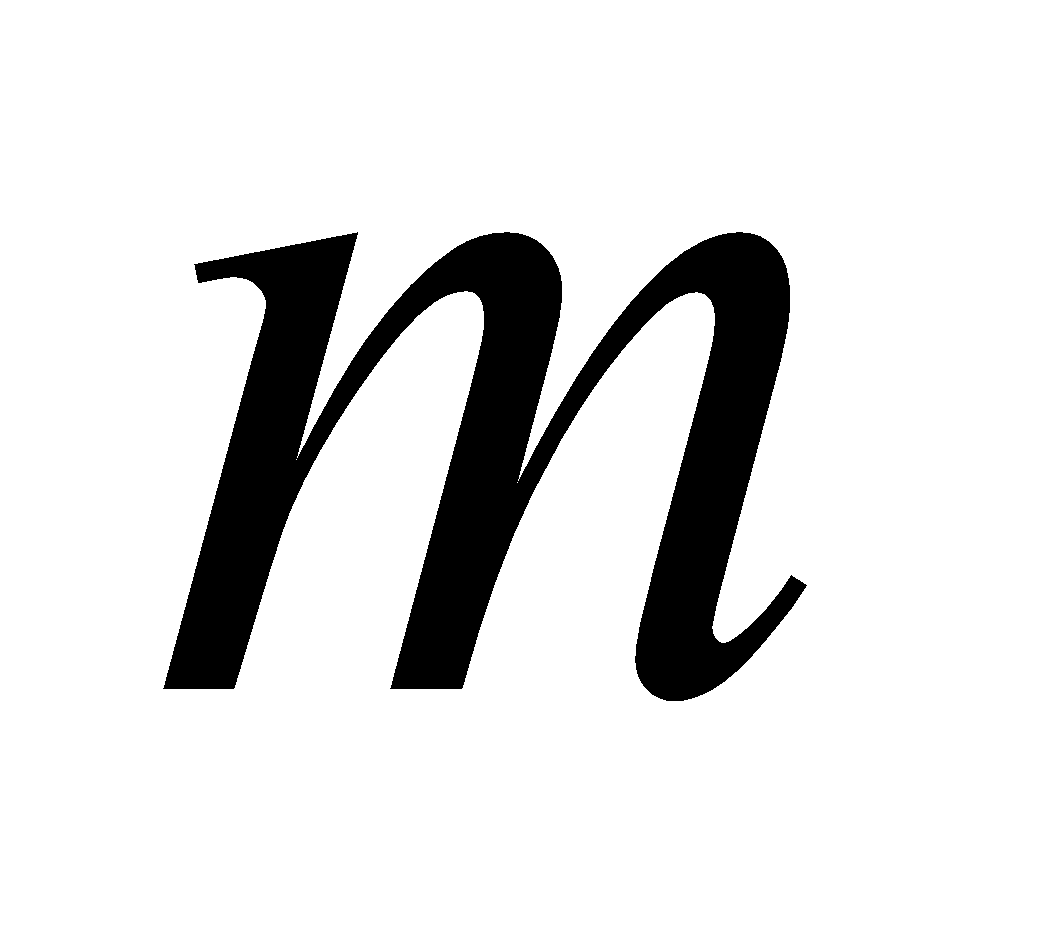are the bounds ofin, then prove that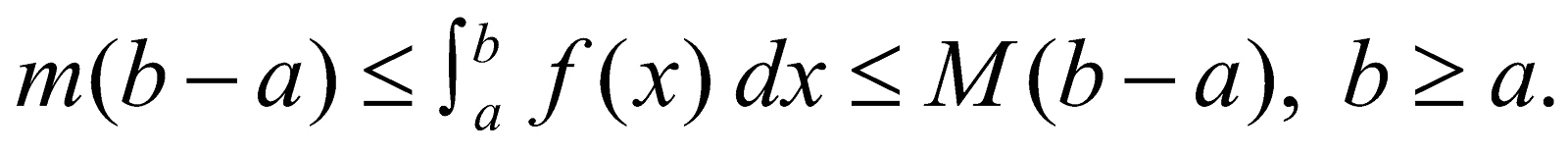3
(c) If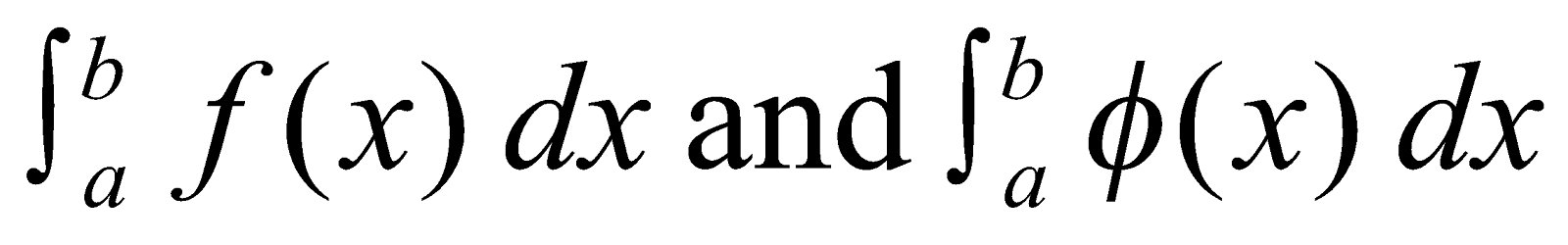Both exist and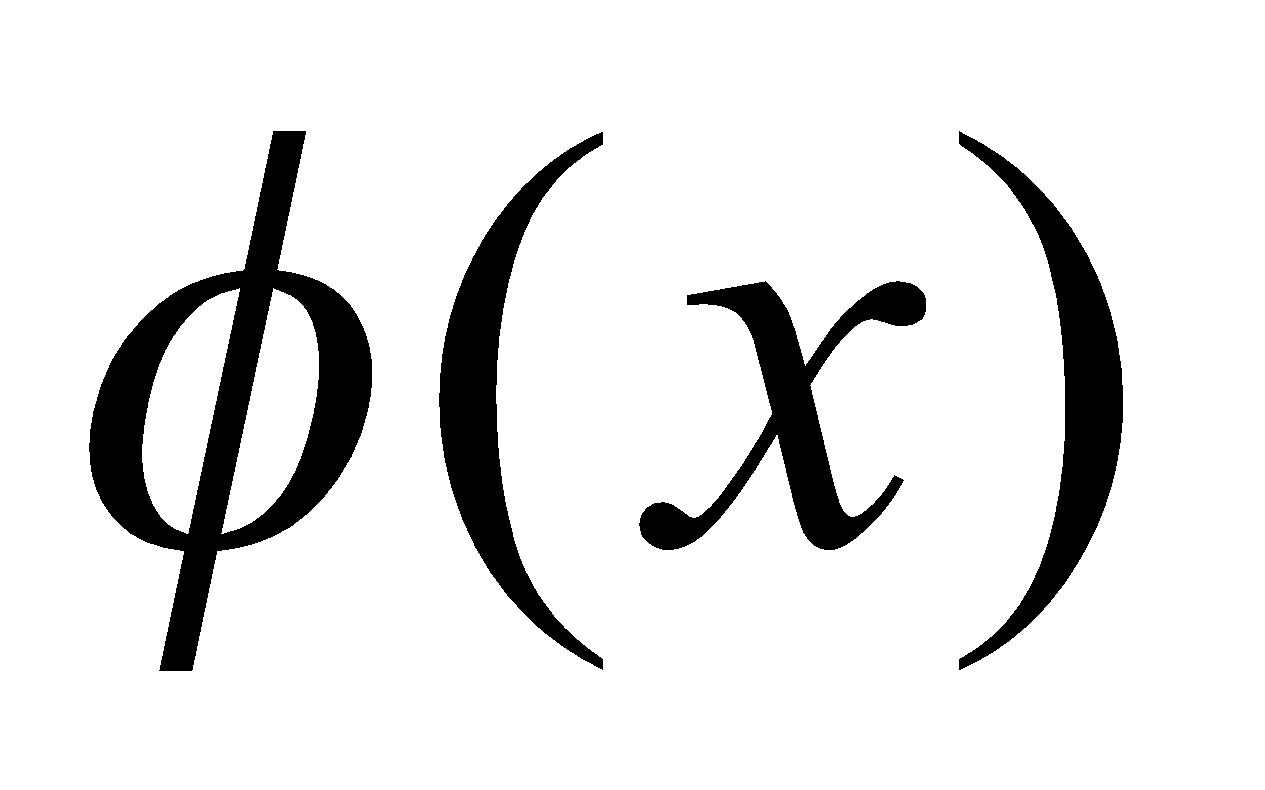keeps the same sign throughout the interval, then prove that there exists a number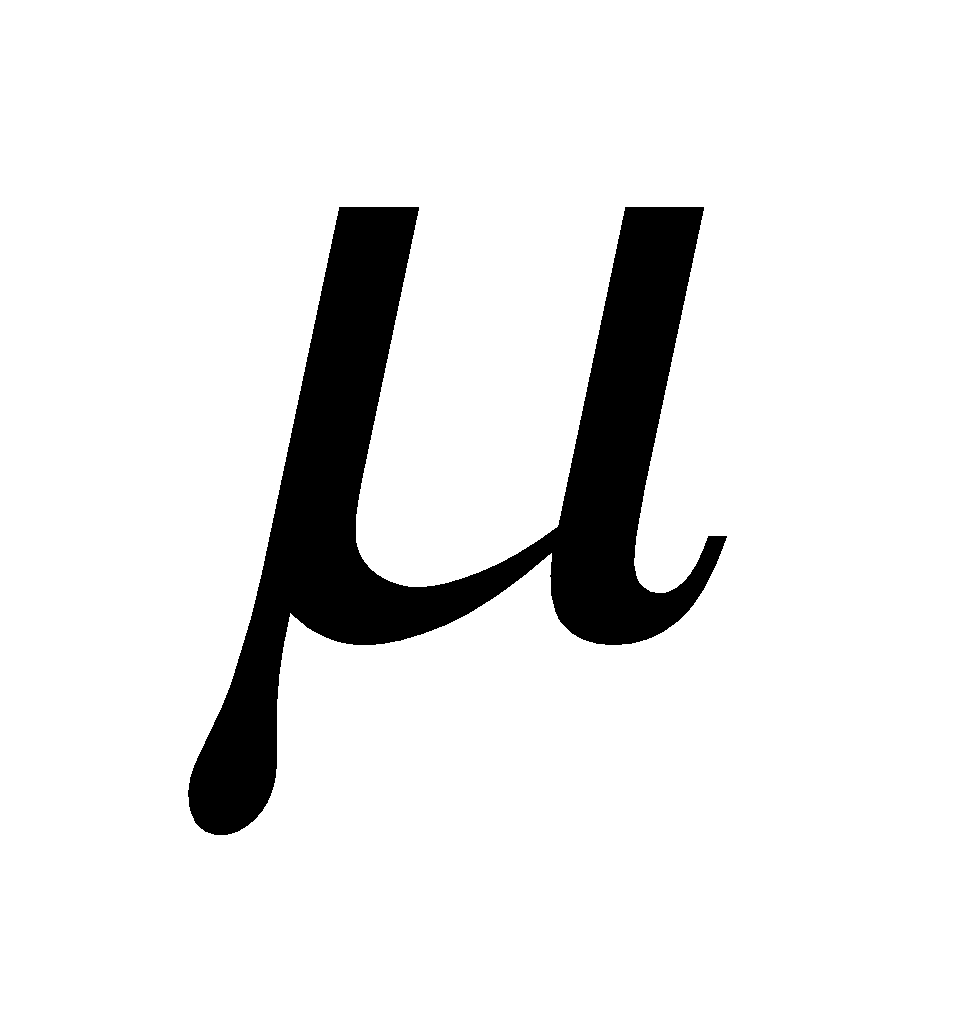between the bounds ofsuch that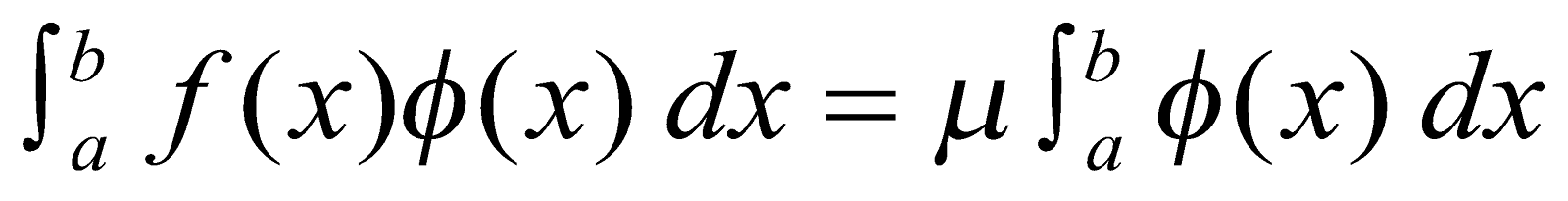3
9. (a) Give example of an improper integral of second kind. 1
(b) Test for convergence of 2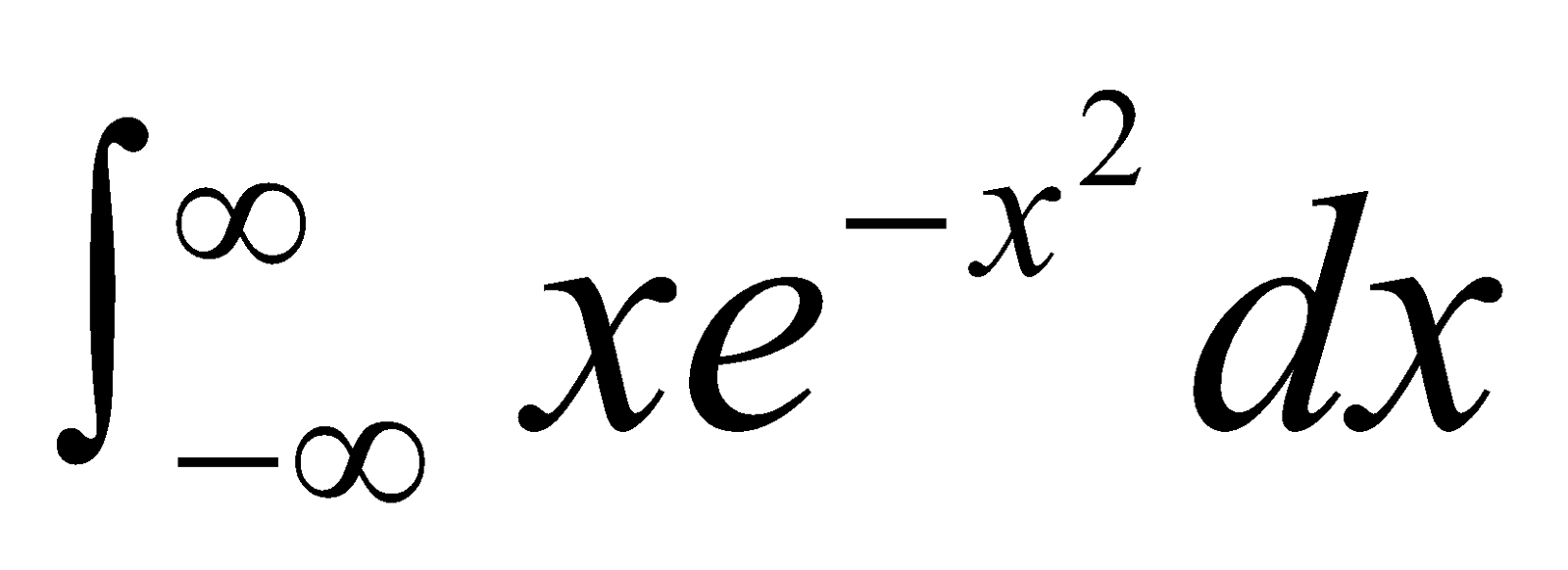(c) Prove that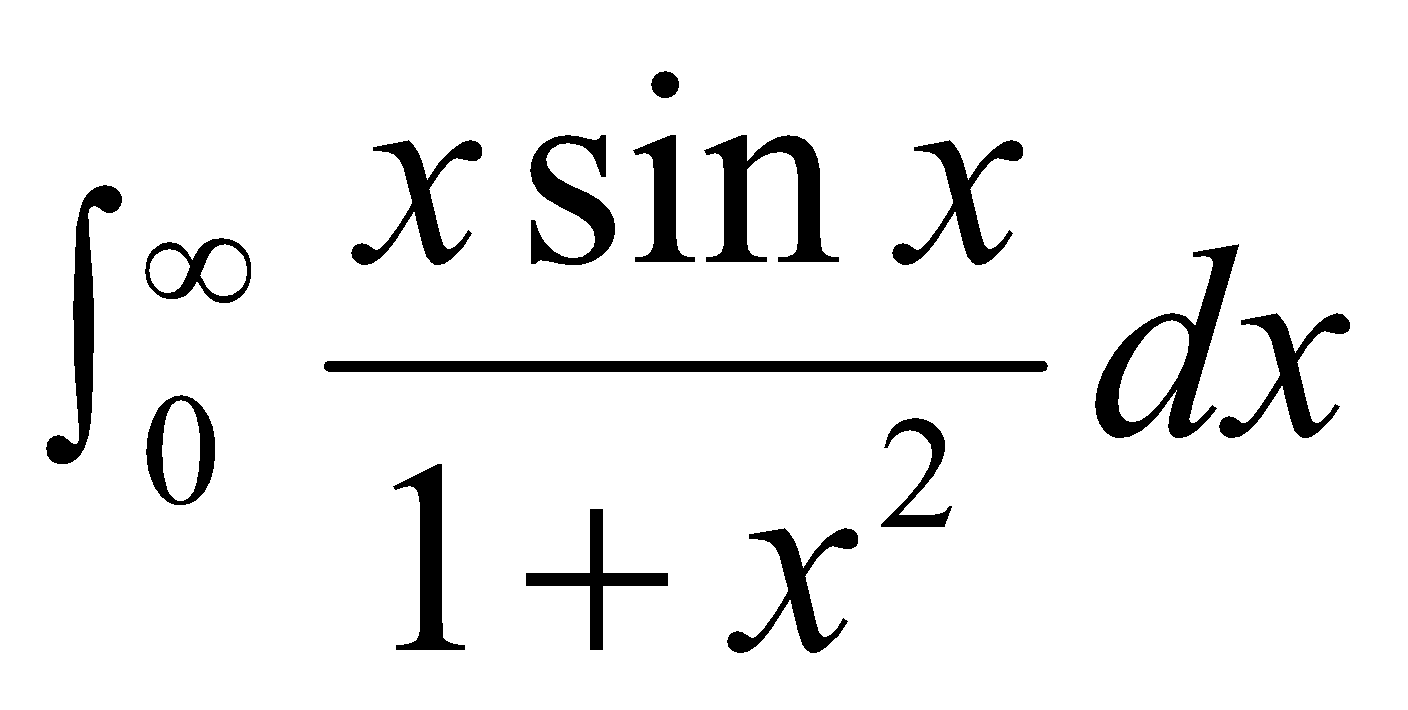converges. 3
10. Answer any one of the following: 4
1. Prove that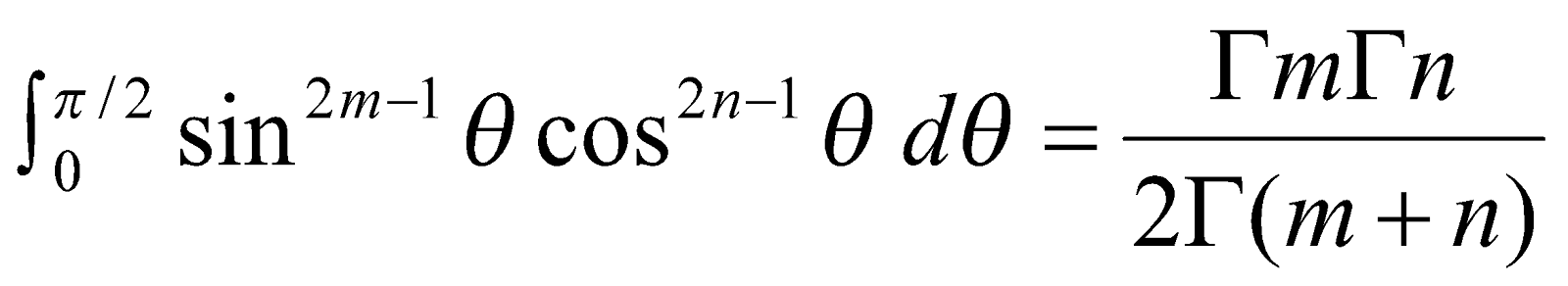Hence deduce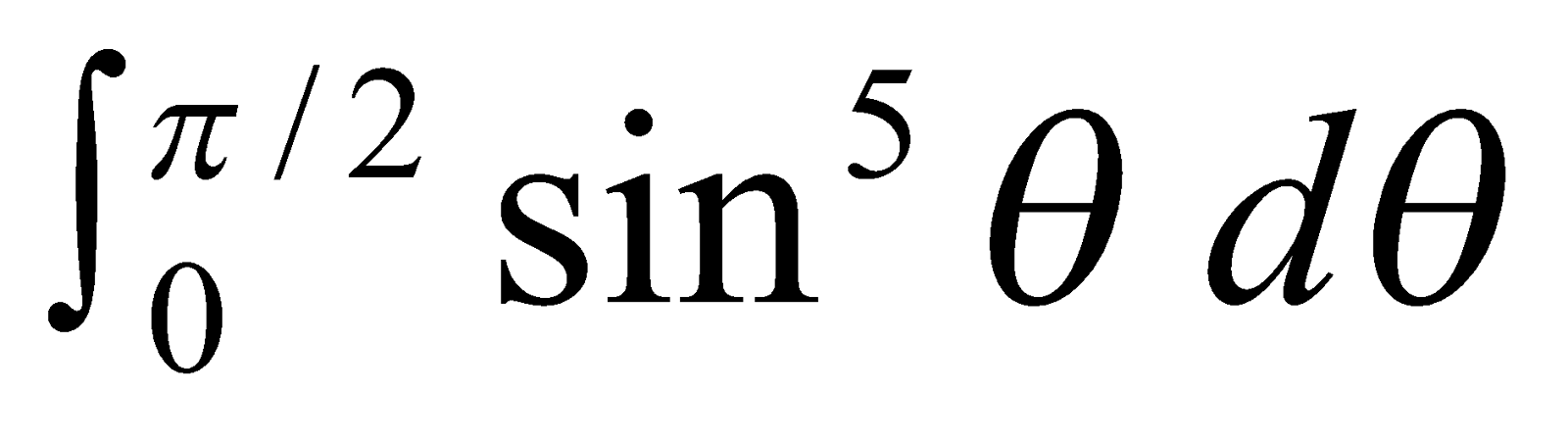1. Prove that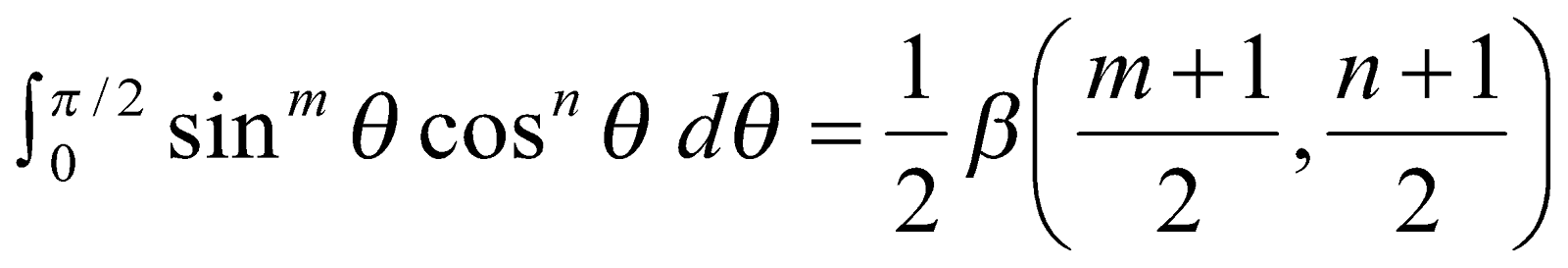and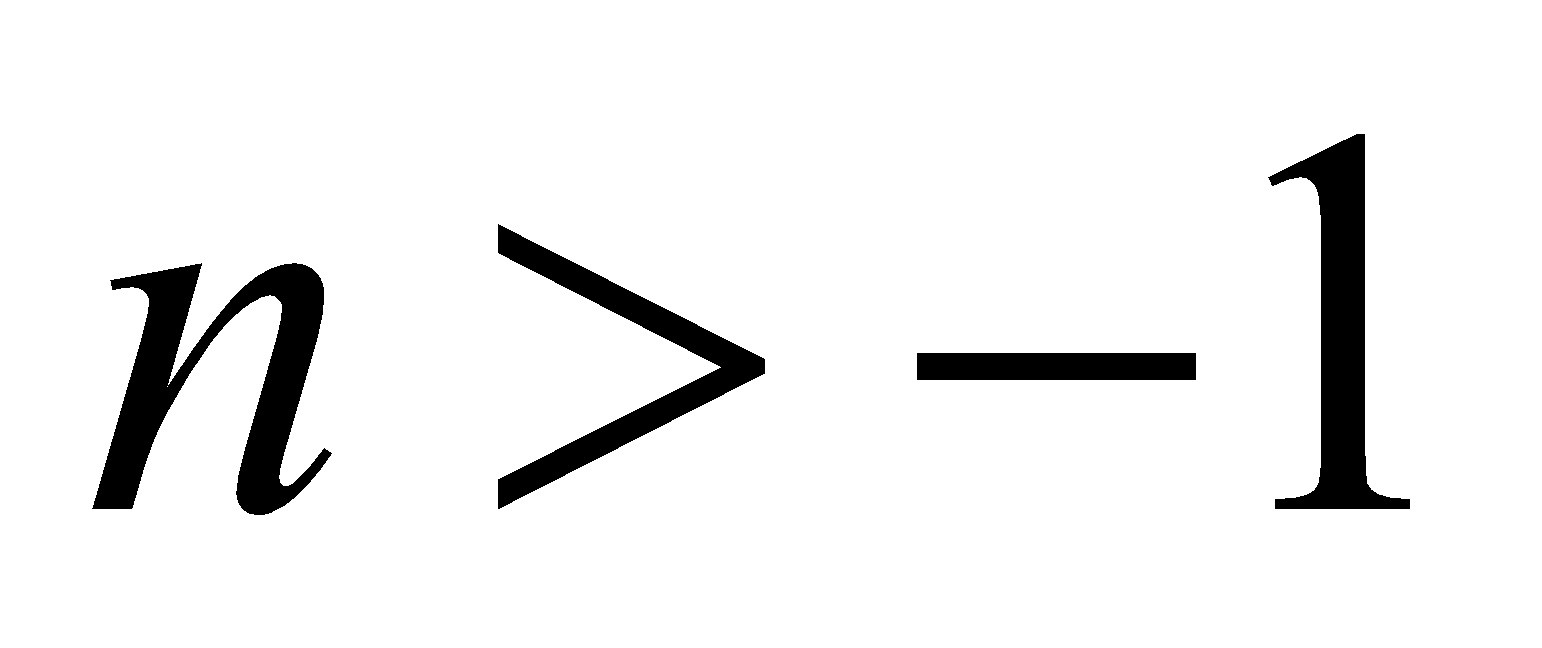.
***